Browse by Topic

# Digital Vintage Sound Modeling: Analog Drums with the Wolfram Language and System Modeler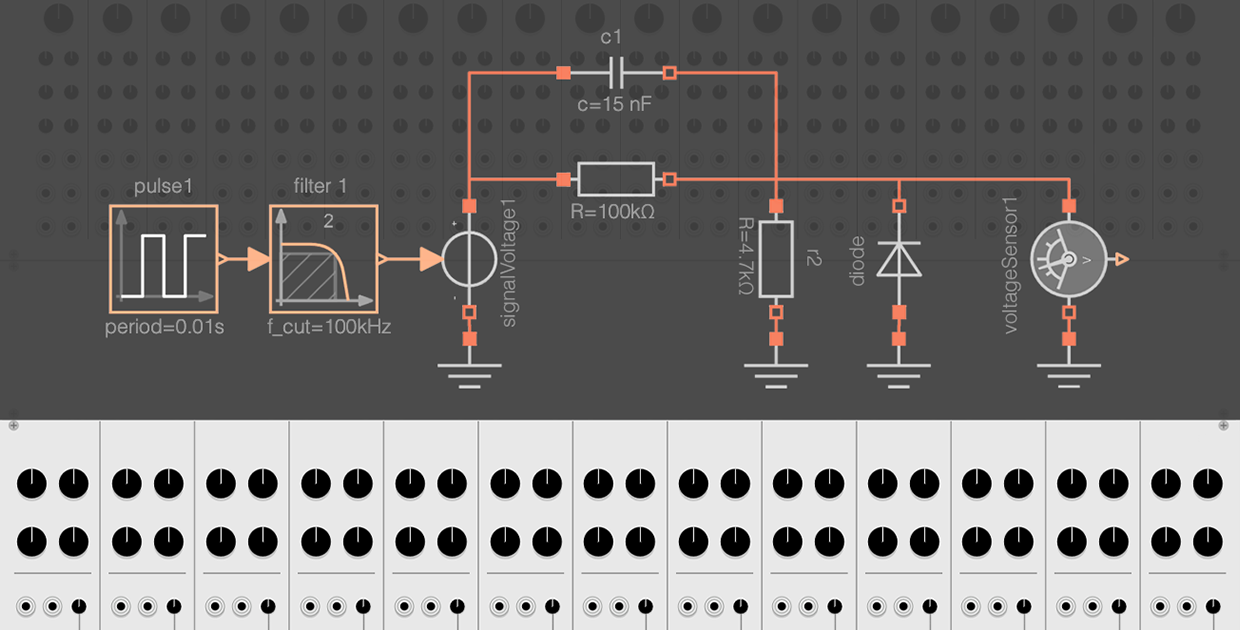Explore the contents of this article with a free Wolfram System Modeler trial.You may not know what a Roland TR-808 Rhythm Composer is, but you have most certainly heard it. The TR-808 is a programmable drum machine released by Roland in 1980. The 808 is one of the most iconic drum machines and has been used in a wide variety of music, such as hip-hop, dance, soul, electro, pop and many more.

The 808 sounds like this: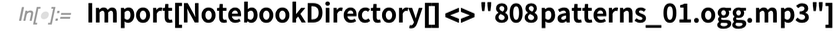✕Every 808 sound has its own personality. For over 40 years, it has fascinated musicians, who have used this drum machine in their music. If you are interested, you can find many videos about the 808, including this trailer for the 808 full-length documentary:

All the 808 sounds are generated using analog electronics. (Other drum machines may use samples, which are recordings of existing drums or percussion.) Over the years, I’ve been analyzing drum synthesis circuits, and the fact that all the drum sounds are generated by simple electronic components made the 808 very intriguing.

In this blog post, we are going to model and simulate the kick drum, one of the most emblematic drums of the 808, using Wolfram System Modeler. We’ll start by creating a component-level model to get an accurate reproduction of the behavior. (Download this zip folder for all the necessary files to experiment with the code in this post.) Later, we’ll simplify and optimize the model to compare the resulting sounds of the different versions against the 808 kick drum hardware.

## Modeling the 808 Kick Drum

Here you can see the schematic I made for the bass drum: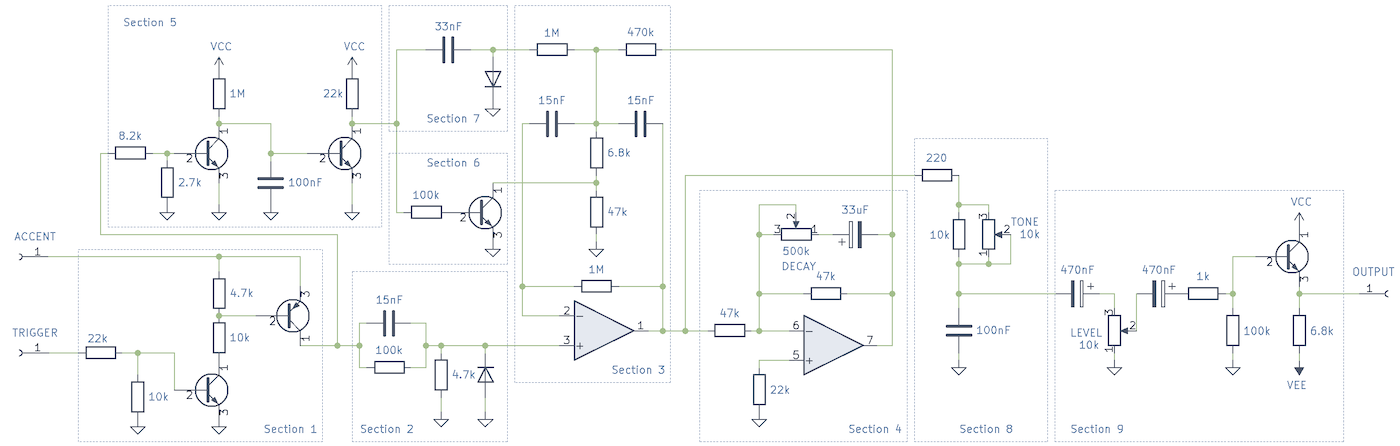✕

(This schematic has small modifications compared to the original, but those modifications are not important for our analysis.)

When trying to understand a circuit like this, I start by looking for small subcircuits or patterns, such as filters, buffers, amplifiers or common operational amplifier circuits. When I’m not sure what the function of a circuit is, I simulate it and also corroborate the results against the real circuit. In this case, I have created my own replica of the circuit we’ll use as a reference: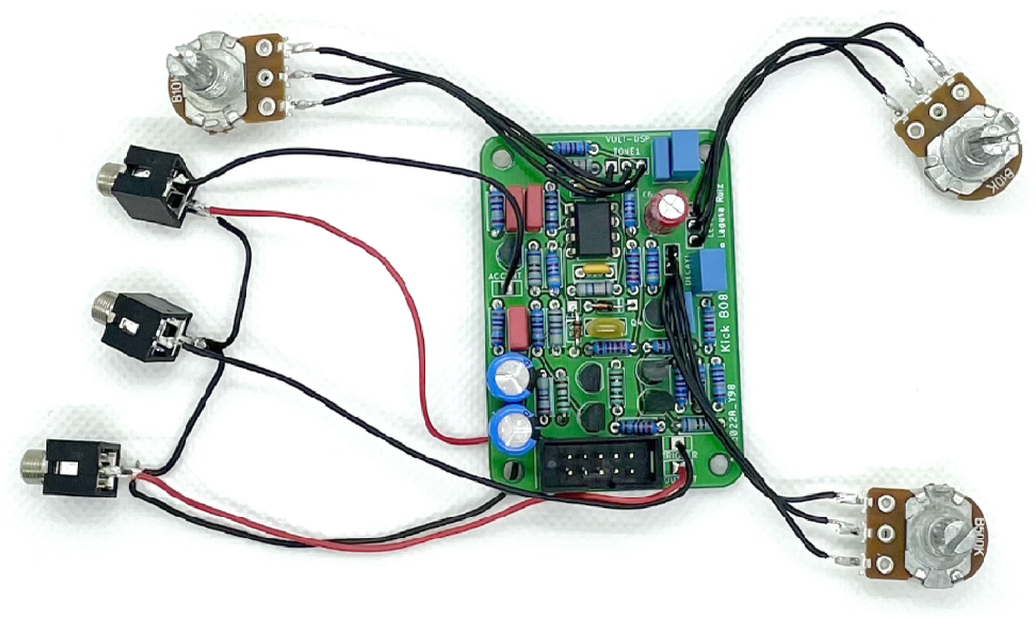✕

## Understanding Each Section of the Circuit

In the previous schematic, I have already divided the circuit into smaller subcircuits. We are going to analyze each of those sections by performing simulations individually, and at the end simulate the full circuit.

As we follow the signal flow, the first parts we will analyze are the processes of the Accent and Trigger signals. We’ll refer to this part of the circuit as Section 1.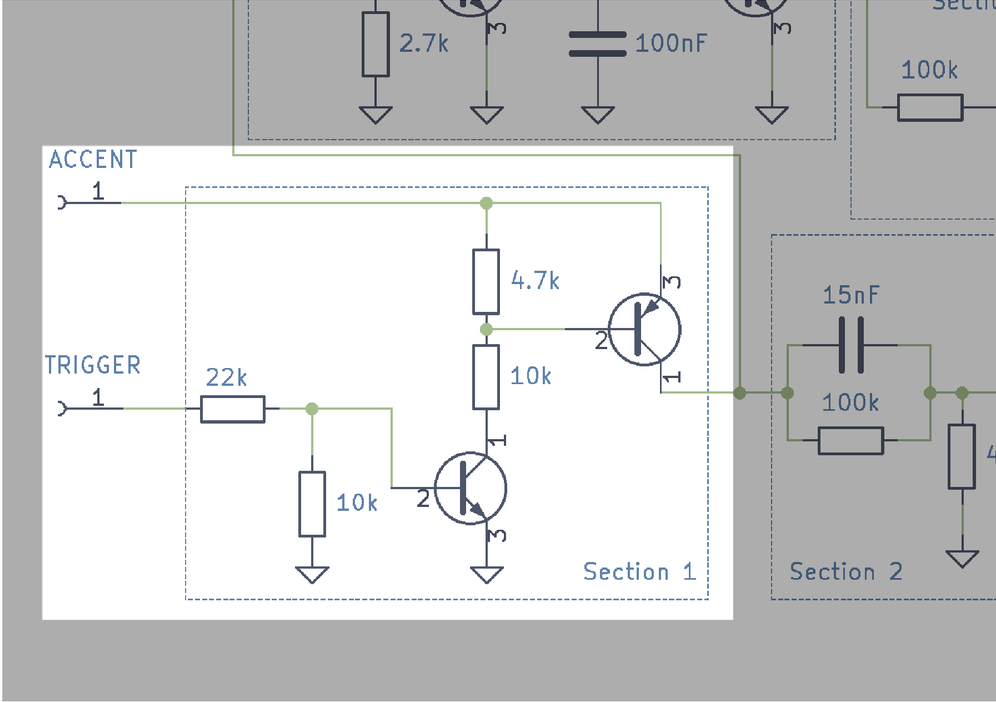✕

Triggers are usually small pulses of around 1 ms that have an amplitude of 5 V. Accent signals define the velocity (intensity) of the drum sound hit. In this drum machine, there are three levels of intensity: a normal hit, an accent note and a ghost note. The voltage of the accent note defines the intensity. A large voltage (e.g. 14 V) will produce an accent note, while 8 V will be a normal note. In a similar fashion, a low voltage (e.g. 4 V) will produce a soft (ghost) note.

The Section 1 circuit consists of two transistors and a few resistors. We can simulate this circuit in System Modeler by using the generic transistor models included in the Modelica Standard Library because, in this specific case, the transistors act as switches. You can see in the following diagram the equivalent circuit made in System Modeler: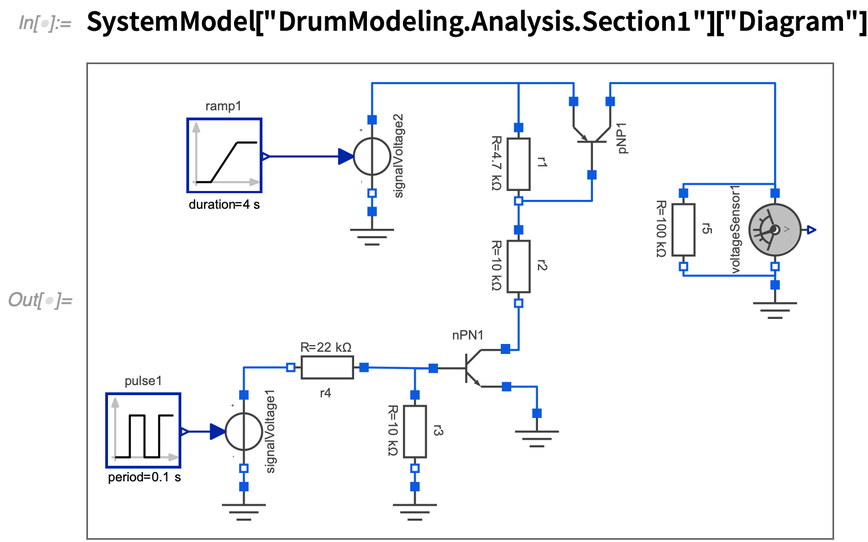✕

In this simulation, the Accent and Trigger signals are generated using the Ramp and Pulse blocks from the Modelica Library. The Accent goes from 0 V to 8 V, and the Trigger produces pulses of 5 V. We can see from the simulation results that when the Accent is above (approximately) 2 V, we get a pulse similar to the Trigger as output but with the amplitude of the Accent signal. This circuit behaves as a controlled switch where the Trigger signal controls the flow of the Accent signal: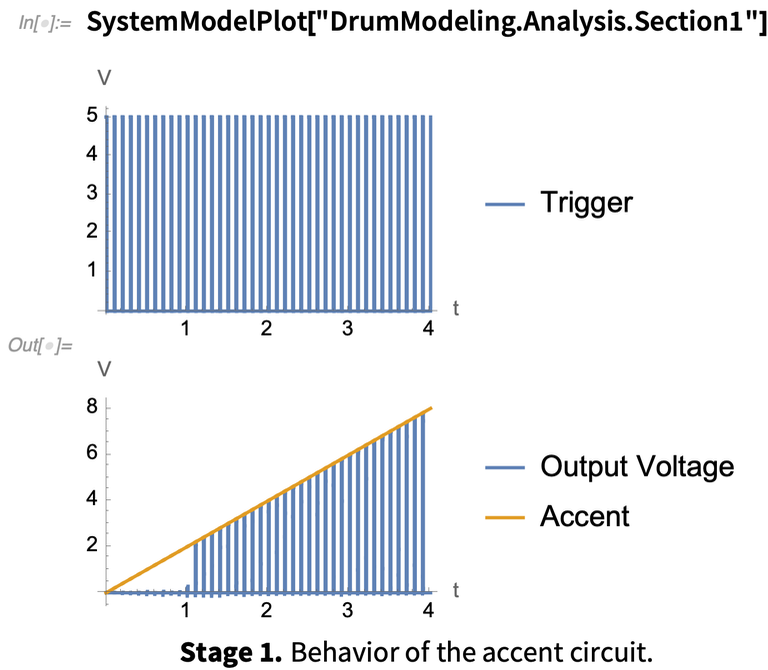✕

We can see in the simulation that the switch is not perfectly linear. The accent needs to be at least ~2 V to get an output.

The next section of the circuit (Section 2) takes as input the pulse produced by the circuit we analyzed previously.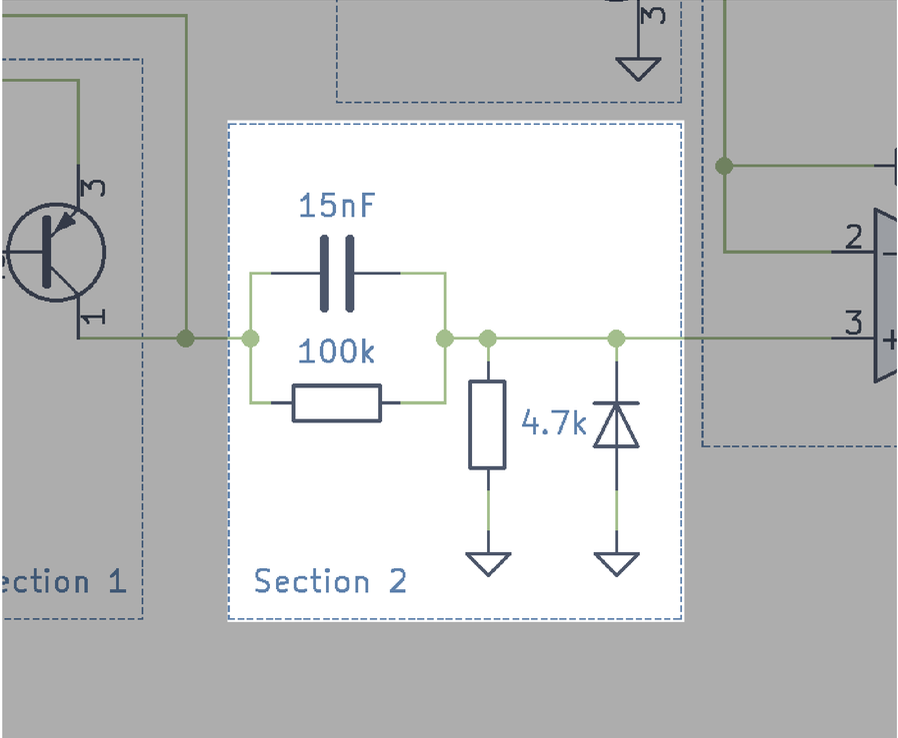✕

This circuit looks like a passive highpass filter, with the exception of the diode. The output of this stage is connected to the positive terminal of the op-amp. Thanks to that, we can assume it is connected to a high-impedance load.

We can recreate this circuit in System Modeler and use a generic diode model: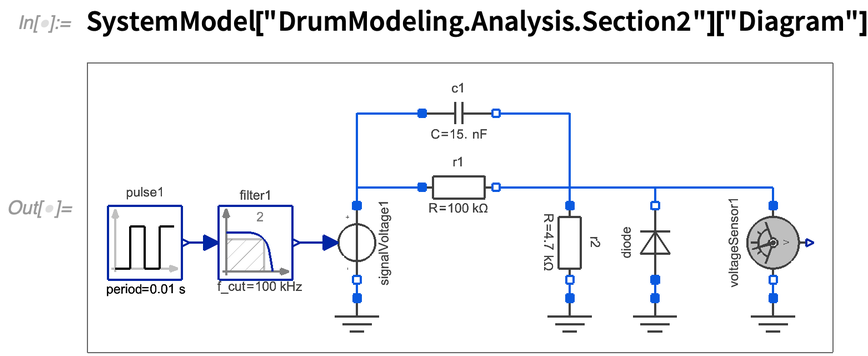✕

If we simulate this circuit using as input a 5 V pulse, we obtain the following results: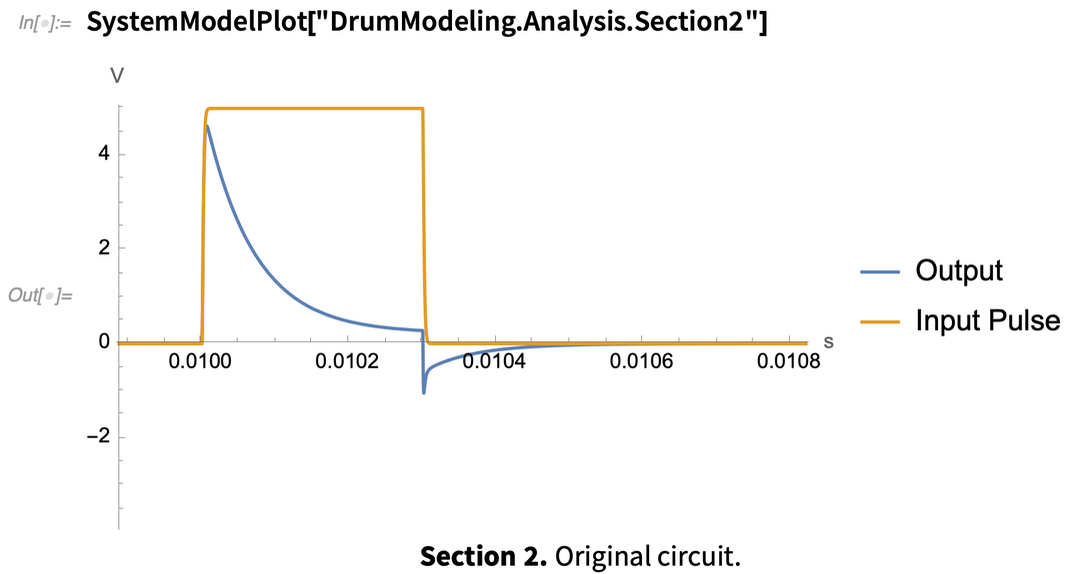✕

When the input pulse jumps from 0 V to 5 V, the output produces a peak that is almost as high as the input. When the input pulse returns to 0 V, the output has a small negative peak. By removing the diode and rerunning the simulation, we can see the purpose of the diode: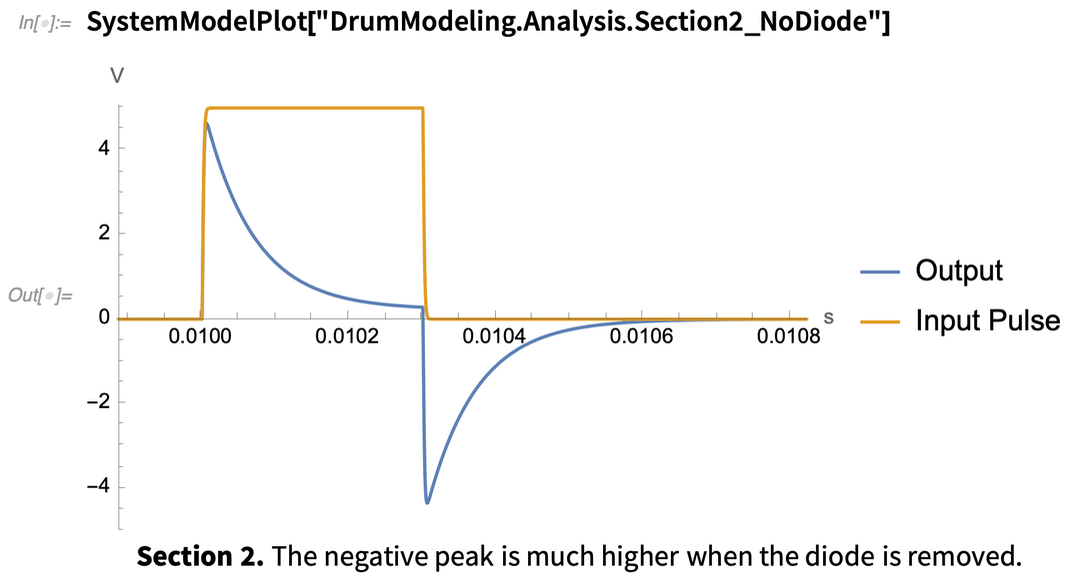✕

We can see a large negative peak in the output. The diode was put there to suppress the negative peak of this signal. The resulting pulse is now sent to the main resonator circuit (Section 3). This is the central part of the drum, and we’re going to analyze it next.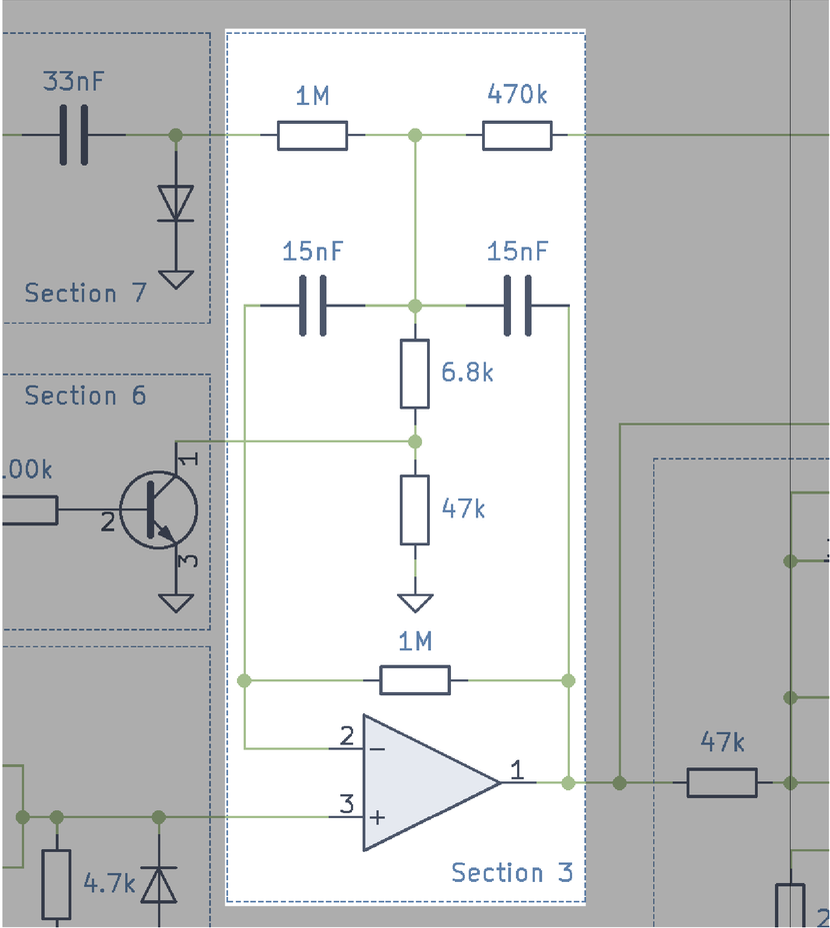✕

This part of the circuit is know as a bridged T-network or multiple feedback bandpass filter.

Let’s start by creating a simplified version of this circuit to analyze it: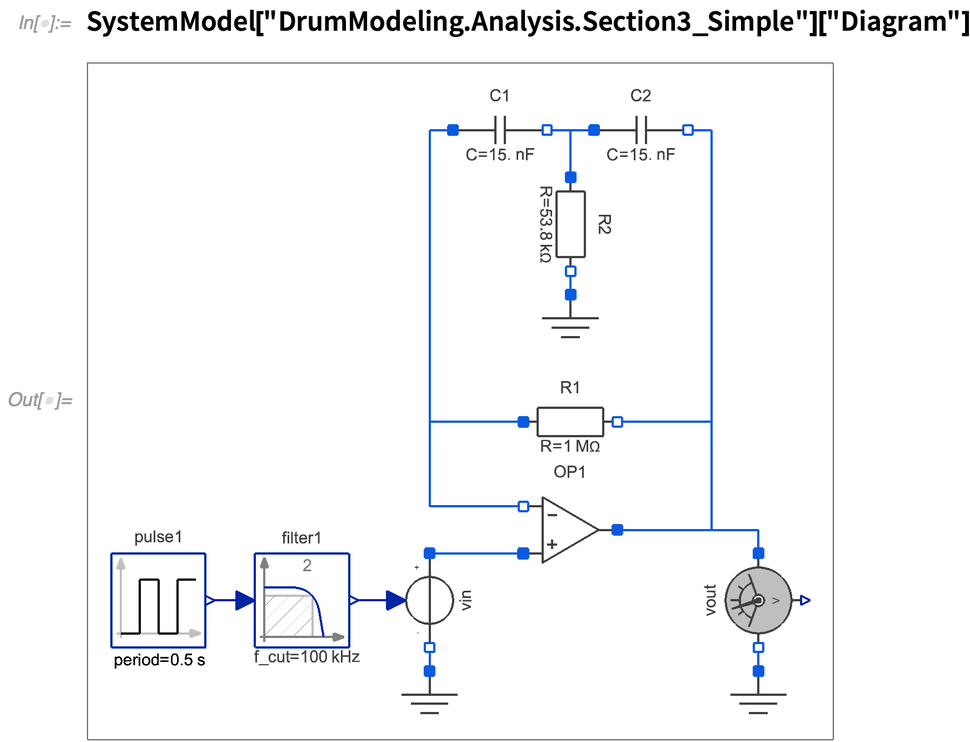✕

In the previous circuit, we eliminated two of the inputs (the top of the original circuit), and the resistors of 47 k and 6.8 k have been combined into a single resistor of 53.8 k. We are using a small pulse of about 1 ms to excite (ping) the filter. The simulation results are displayed in the following plot: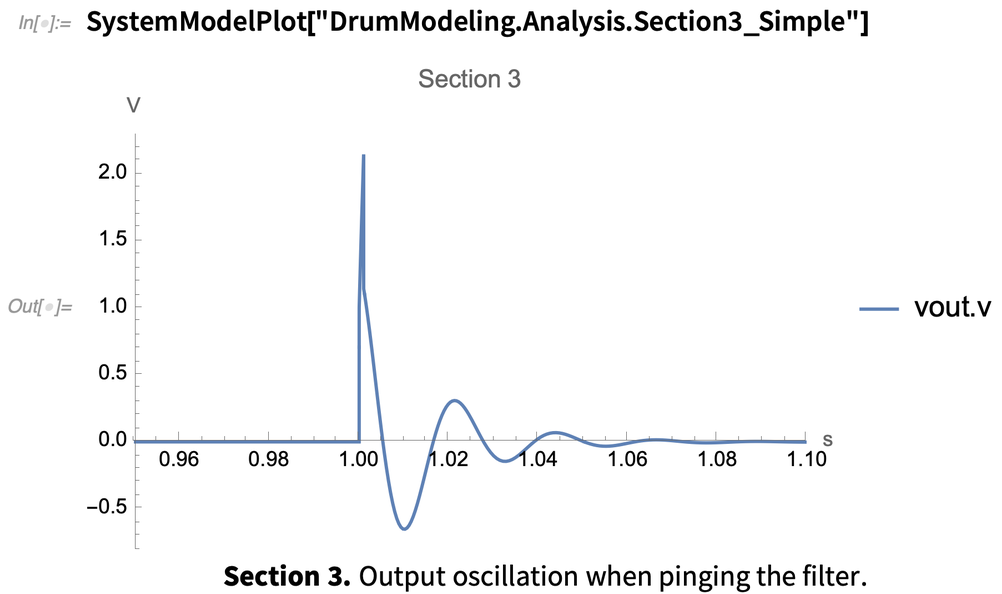✕

We can see from the results that introducing a small pulse in the filter makes it oscillate (resonate) for a short time. The frequency of this oscillation is defined by the central frequency of the bandpass filter, which is defined by the values of the capacitors and resistors C1, C2 and R2 in our circuit.

You may remember that in this simulation, R2 in the original circuit is comprised of two resistors (47 k and 6.8 k), as shown in this circuit: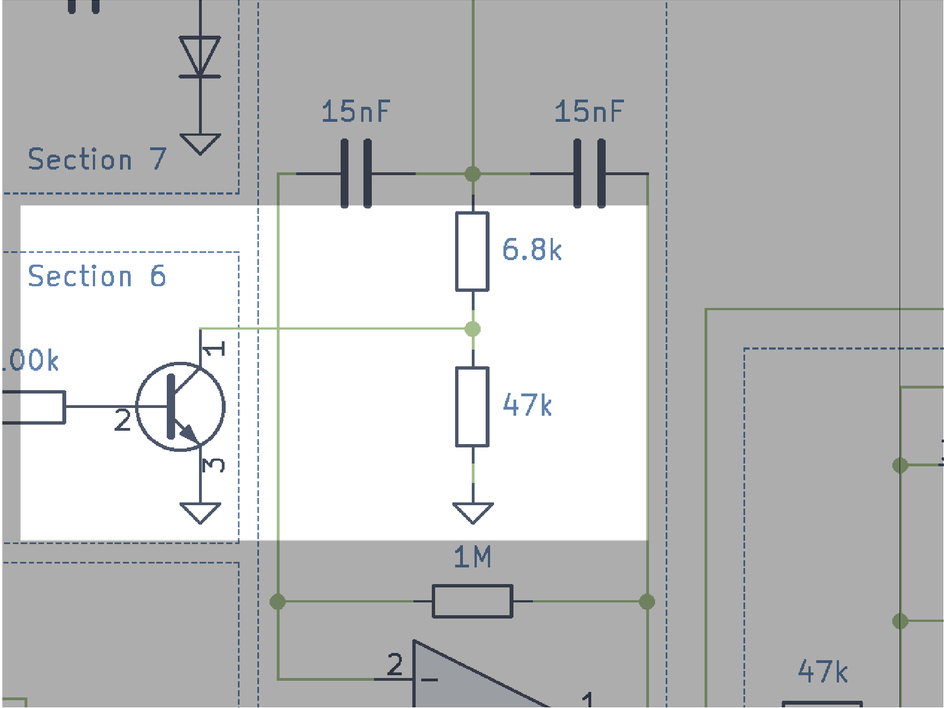✕

You may also notice there is a transistor connected in parallel with the resistor of 47 k (Section 6). This transistor seems to be operating as a switch that is used to short-circuit the resistor of 47 k. As mentioned before, changing this resistor value will make the filter resonate at a different frequency. In the following plot, we can see the results of short-circuiting the 47 k resistor, which changes the effective resistance from 53.8 k to just 6.8 k: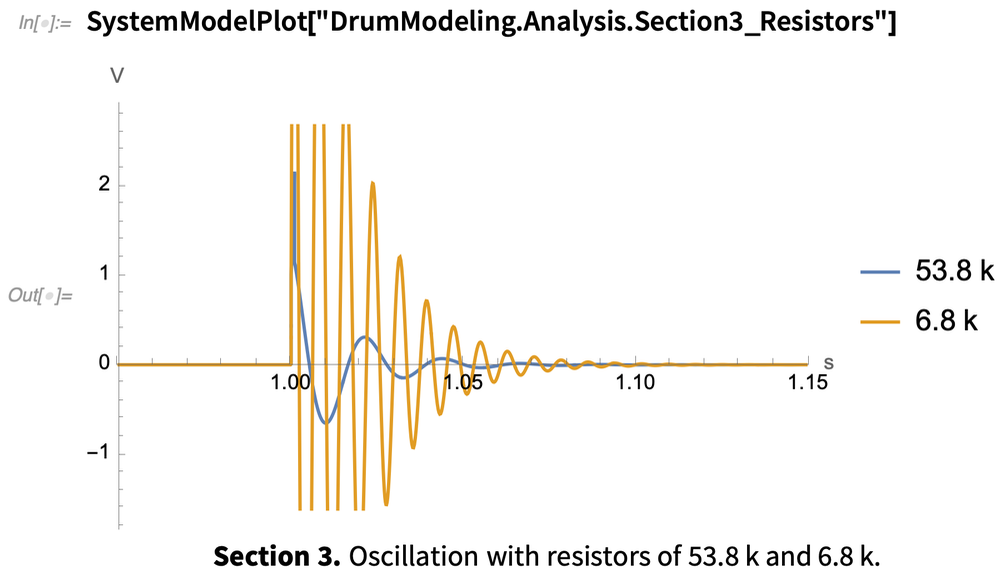✕

With a resistor of 6.8 k (47 k in short-circuit), the filter resonates at a higher frequency. A signal controls the switch (transistor in Section 6), but we are going to take a look at it later. Just remember that this signal is used to change the frequency of the filter.

At this stage, we can already listen to the drum sound by simulating our model (with the 6.8 k resistor). Note that you may need headphones or a decent sound system to listen to the low frequencies: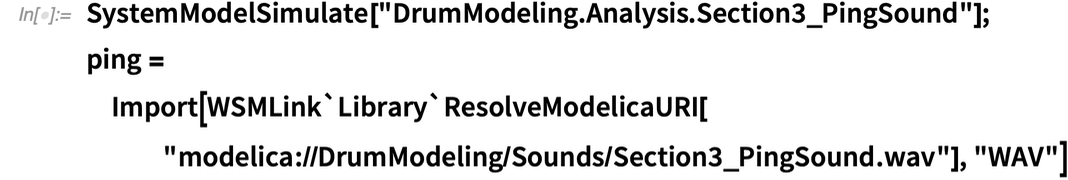✕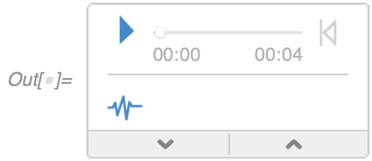After the resonator, there is an operational amplifier that includes a potentiometer labeled as “Decay”. This is our Section 4, which is shown in this circuit: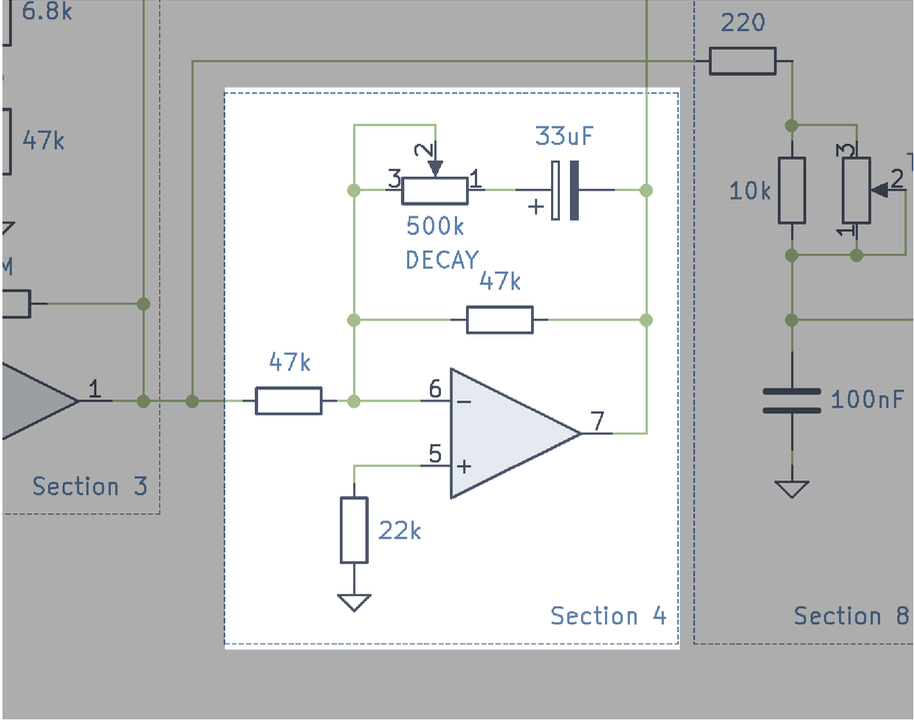✕

In the drum context, “decay” refers to the amount of time the drum produces sound. A short decay sound is a fast sound, for example the sound produced by knocking a door. A long decay sound would be like strumming the string of a guitar, which usually takes a few seconds to stop making sound. Based on the potentiometer description, we can assume that this stage is in charge of controlling the decay of the drum.

This section is comprised of an operational amplifier working on inverting mode. If we ignore for a moment the 33 uF capacitor, we can see that we have the 500 k potentiometer connected in parallel with a 47 k resistor. The equivalent resistance of these two can be calculated with this formula: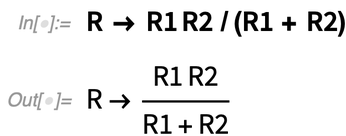✕

In our case, the resistance of the potentiometer varies from 0 k to 500 k ohms depending on its position. Based on the previous formula, we can calculate the equivalent resistance in function of the position of the potentiometer (pos):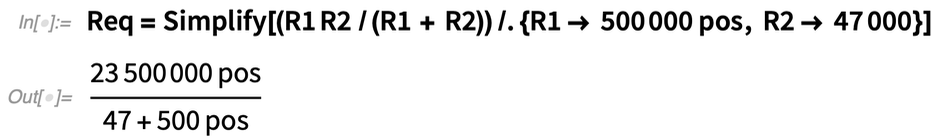✕

If we plot the equivalent resistance in function of the potentiometer position, we can see that this behaves as a logarithmic potentiometer, where its value goes from 0 k to ~42 k ohms: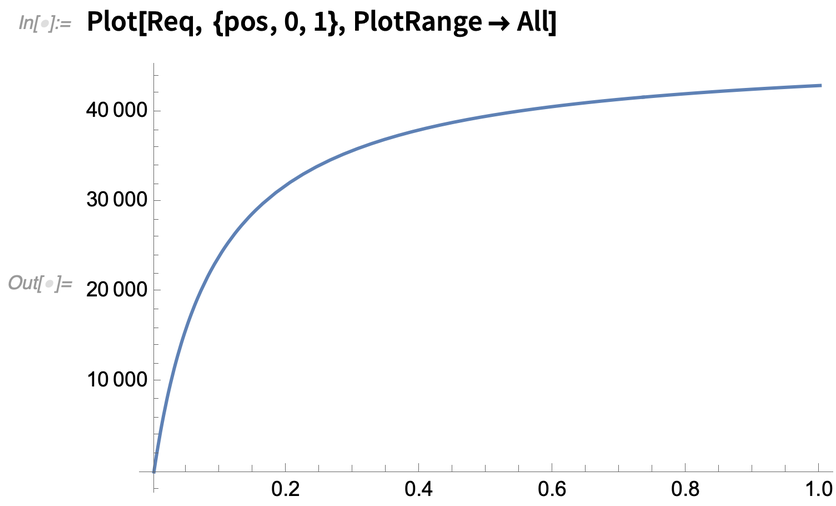✕

As mentioned earlier, the operational amplifier is connected as an inverting amplifier. The formula for the gain of an inverting amplifier is given by the ratio of the feedback resistance over the input resistor. In our case, the feedback resistor is the equivalent resistance (Req) and the input resistor is 47 k; therefore, the DC gain of Section 4 is as follows: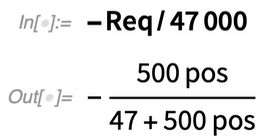✕

Let’s perform a few simulations to corroborate the previous analysis and to also find out the purpose of the 33 uF capacitor in the feedback. In the following figure, you can see a slightly simplified version of Section 4. The only change is that we have dismissed the resistor connected to the positive input of the op-amp and fixed the position of the potentiometer to 10% (0.1):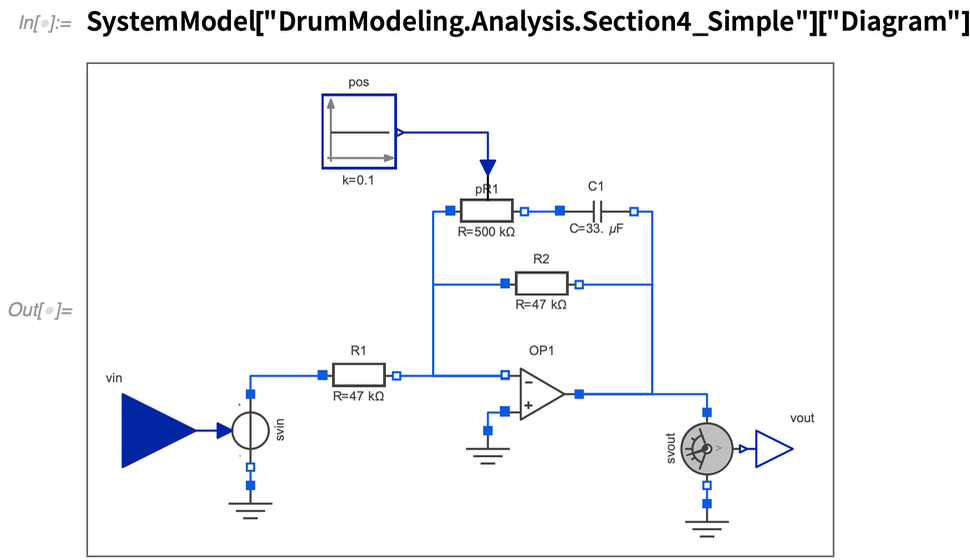✕

Because this model has one input and one output, we can easily linearize it and create a Bode plot to analyze the frequency response: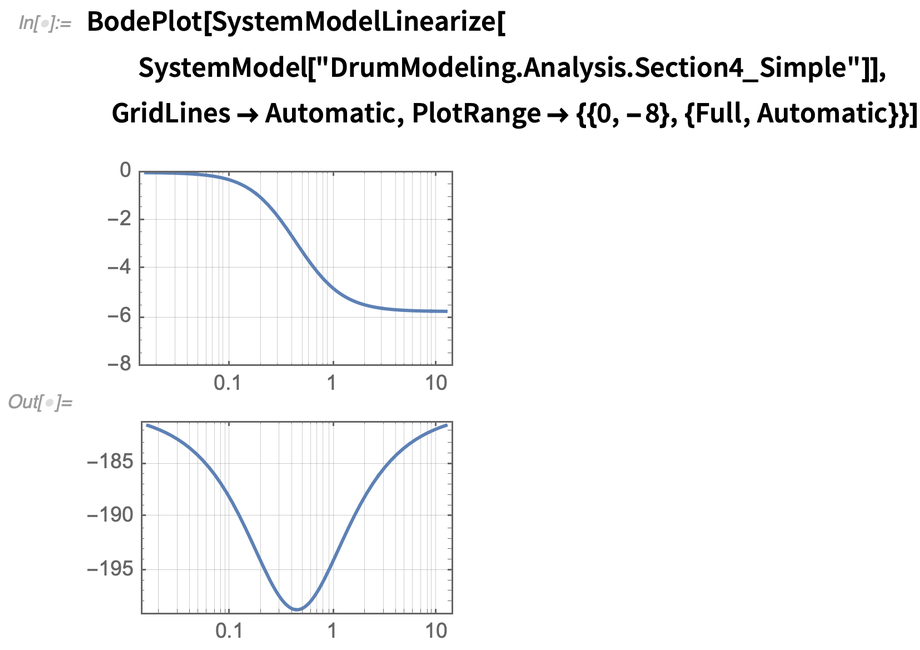✕

From these results, we can observe that for very low frequencies (less than 0.1 rad/s), this circuit has a gain of 0 dB. For frequencies larger than 1 rad/s, the gain is given by the formula we calculated before. Basically, the 33 uF capacitor is there to let the DC pass unaffected, but attenuates all other frequencies according to the position of the potentiometer.

The output of Section 4 is connected (as feedback) to Section 3. This section is shown in the following figure. Let’s make a simulation model and test the effect of the decay: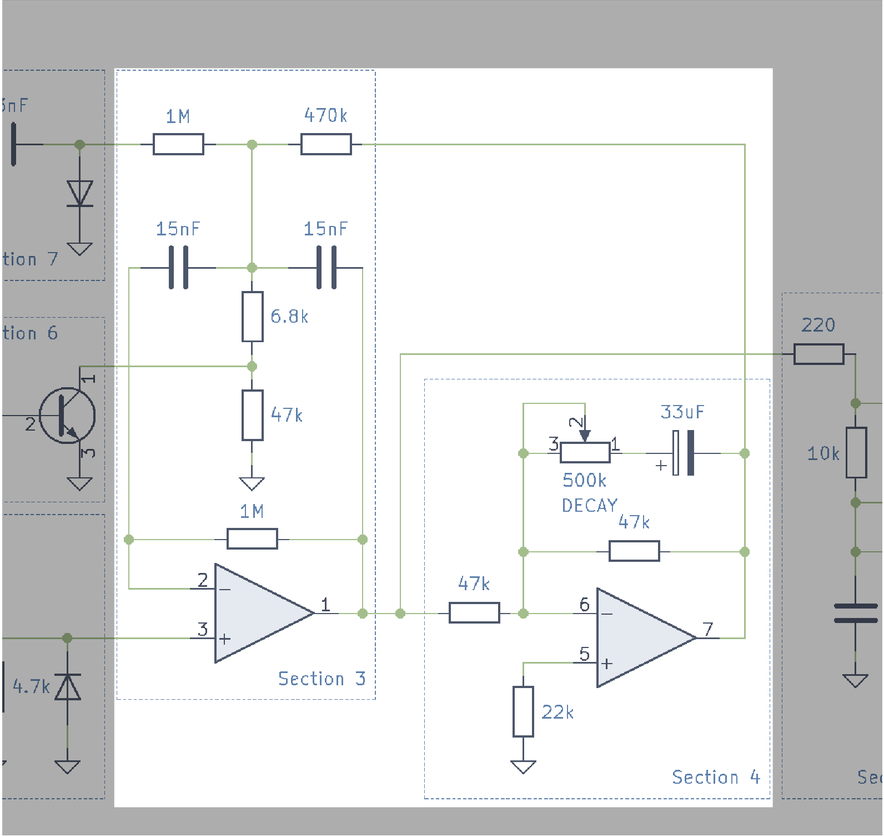✕

In the next figure, you can see the simplified simulation model in which I have removed the 33 uF capacitor in Section 4. The decay potentiometer is set to 90%: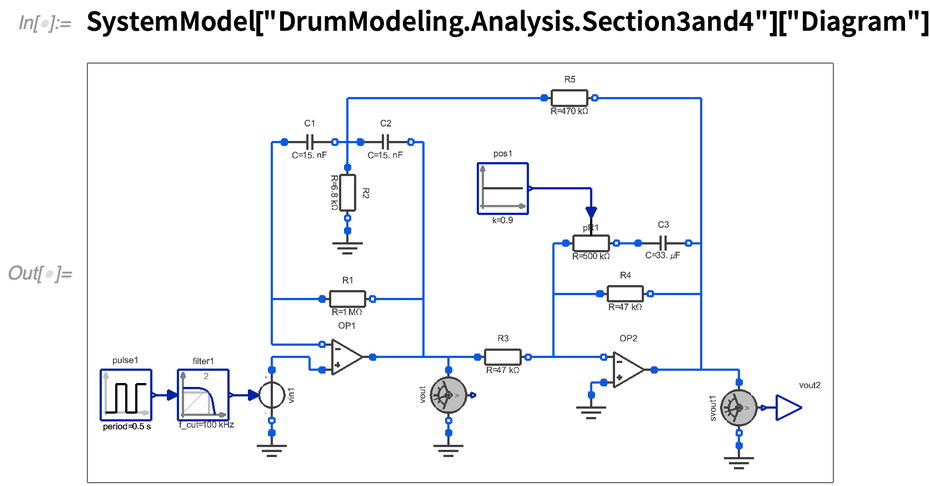✕

Now you can see the simulation results. In this case, the system resonates for a longer time and the next hit is triggered even before the drum stops producing sound: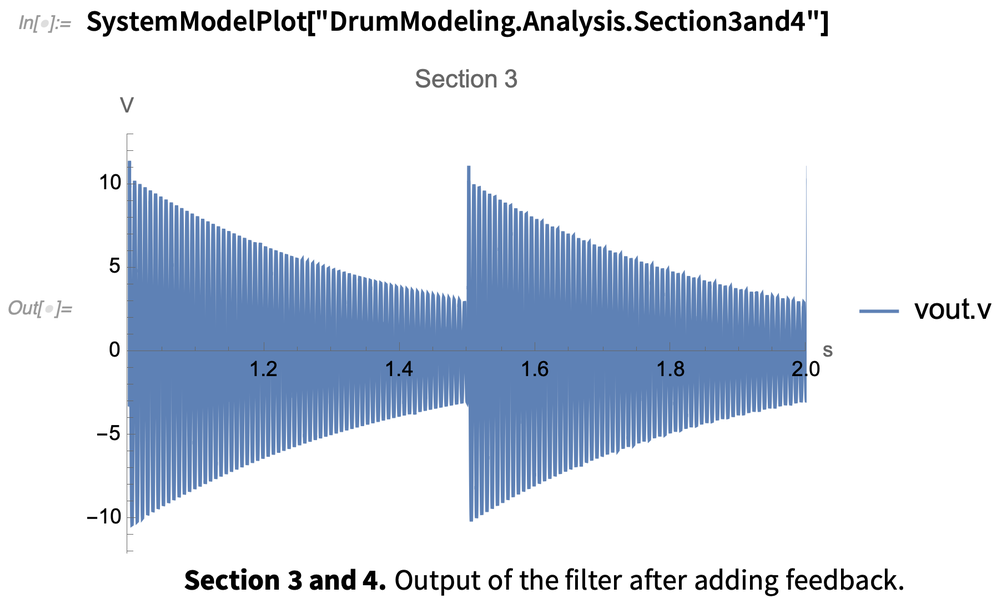✕

By listening to the resulting sound, you can hear that it indeed has a longer decay: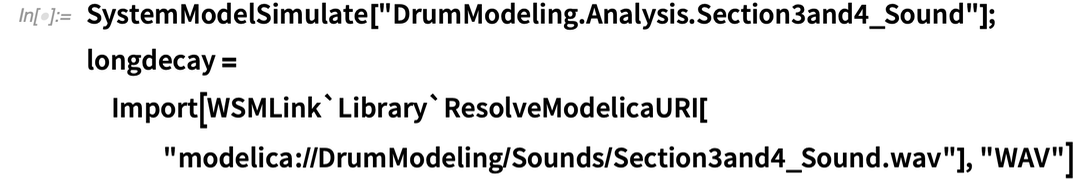✕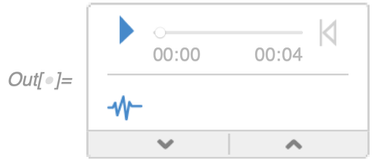So far, we have modeled a part of the main filter (resonator). To continue, we will delve into Section 5: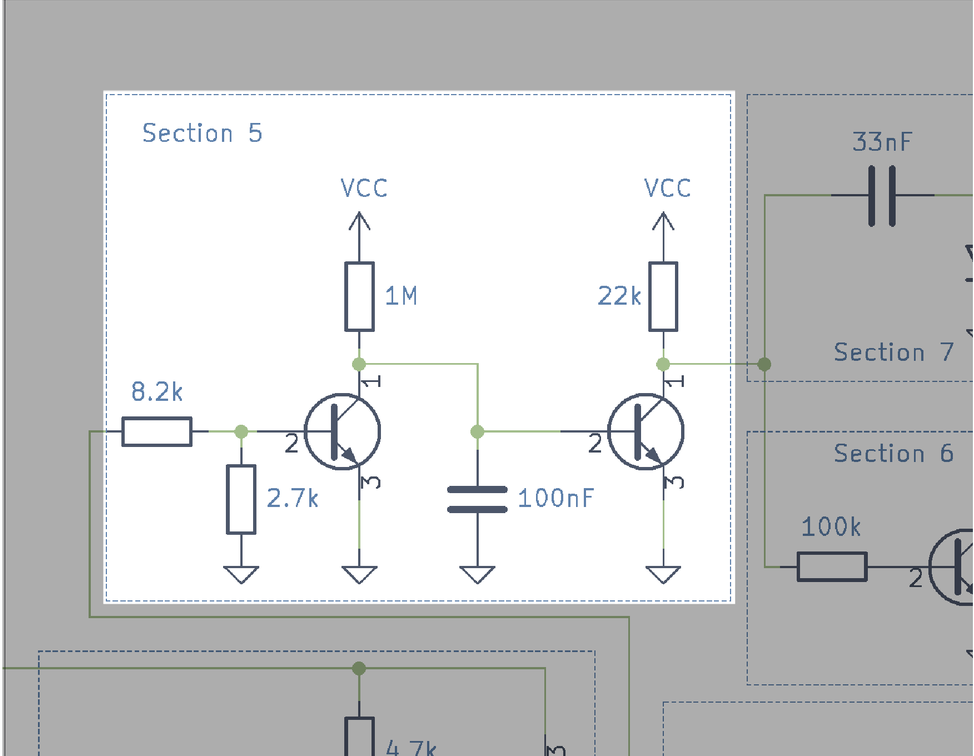✕

Section 5 uses two transistors that seem to be operating as switches. The first one takes the output of Section 1 (the Trigger signal). When the switch is closed, it quickly discharges the 100 nF capacitor. When the switch is open, the capacitor is charged through the 1 M resistor using the VCC voltage (around +15 V). The voltage of the capacitor controls the second transistor. When the voltage in the capacitor is low (discharged), the collector of the transistor (pin 1) is set to VCC through the 22 k resistor. As soon as the voltage of the capacitor polarizes the base of the second transistor, the lower terminal of the 22 k resistor is connected to ground.

Let’s simulate the circuit to have a better view of what is happening: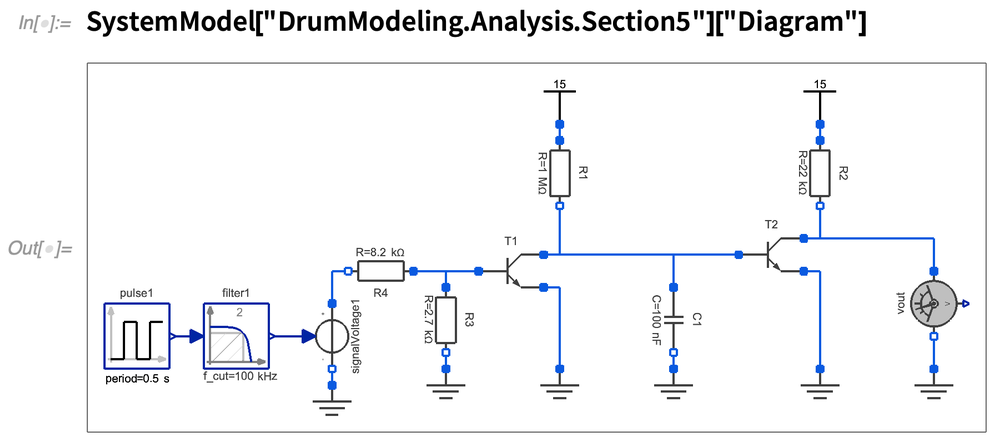✕

In this simulation, we are using as a trigger a pulse of 5 V with a duration of 1 ms. In the simulation results, we see how the capacitor discharges when the pulse is high. The capacitor takes around 6.3 ms before it closes the transistor T2, bringing the output to 0 V. In summary, Section 5 produces a pulse that is about 5.3 ms larger than the Trigger input. This signal is used by Sections 6 and 7: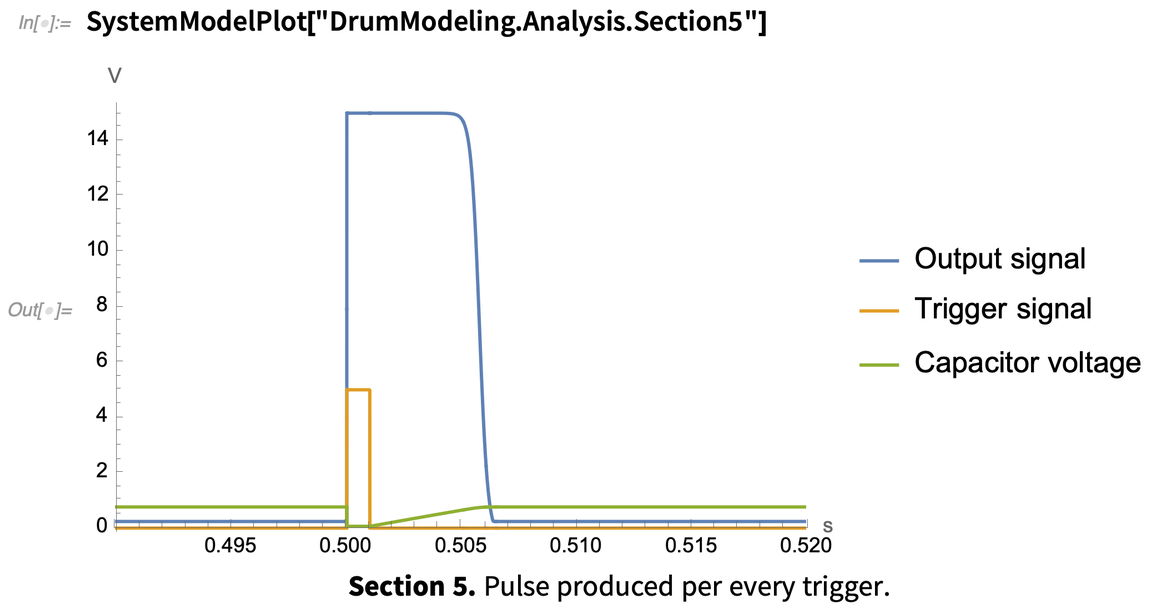✕

The drum machine service manual mentions that this pulse is intended to be 4 ms, but in our simulation we get about 5.3 ms. This difference could be because we are taking a generic NPN transistor model that does not match the original transistor used. It is also important to consider that the real capacitors, transistor and resistors have variability, and when combining these three components, the timing is most likely to be off. In our simulation model, we can easily match the timing by reducing the 1 M ohm resistor.

We have seen previously that the transistor in Section 6 bypasses the 47 k resistor of the main resonator, making it oscillate faster. Because the transistor is controlled by the pulse produced by Section 5, we can conclude that the drum oscillates faster for a short period and then returns to its main oscillation frequency: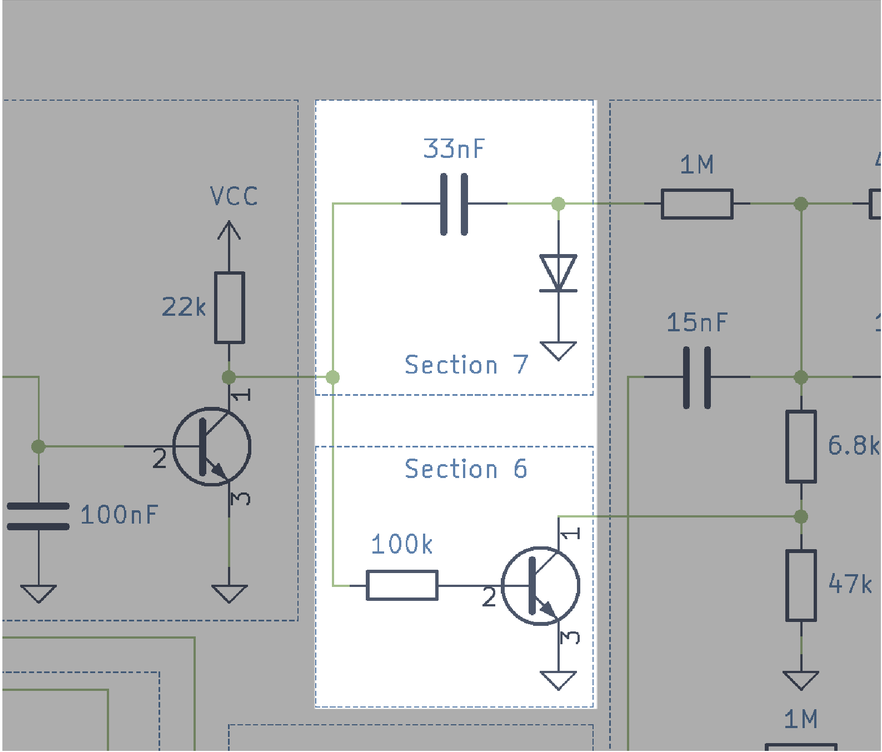✕

That change of pitch in the resonator is there to emulate what happens in a real kick drum. When hitting a real kick drum with a mallet, the membrane of the drum is stretched, which increases the tension, making the pitch higher for a very short period of time. After a few vibrations, the drum returns to the original tension and continues vibrating until the energy is dissipated.

Section 7 receives the pulse of Section 5 too. Let’s create a simplified circuit and simulate it to understand what’s happening: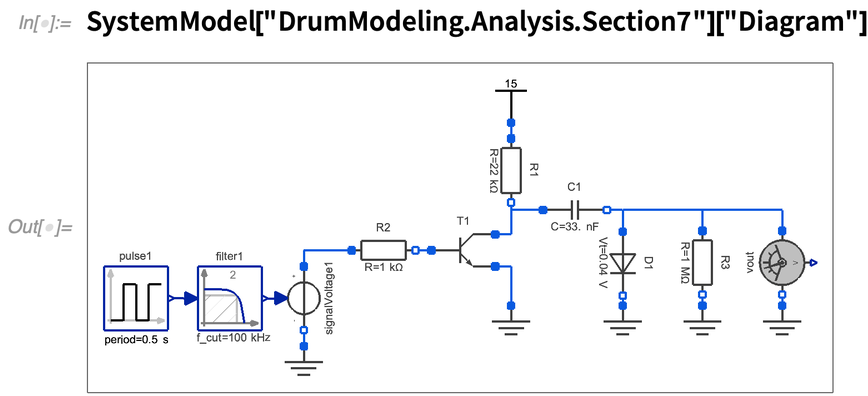✕

In the previous circuit, we recreated Section 7 plus an emulation of the pulse produced by Section 5. In the following plot, you can see the simulation results. This circuit produces a negative pulse with a similar width as the input pulse. The way this circuit works is that when the transistor T1 is open, the capacitor C1 is charged through R1 and D1 to +15 V, and when the transistor is closed, it connects the positive pin of the capacitor to ground. This applies the inverted voltage of the capacitor (–15 V) into D1 and the output. This signal is used to excite the resonator in addition to the trigger pulse: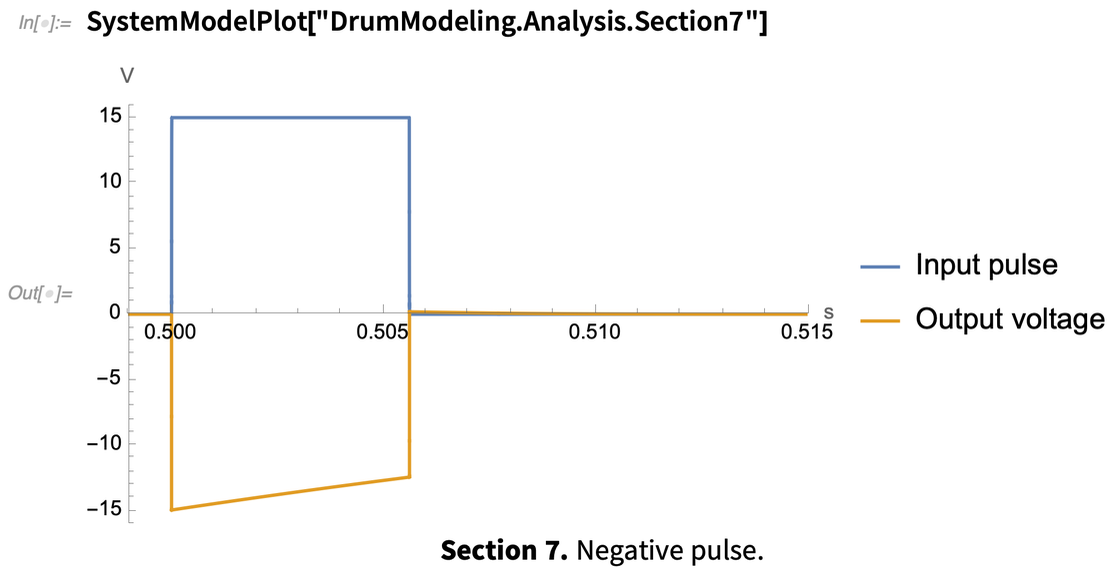✕

The last stage we are going to analyze is Section 8. We are not going to cover Section 9 because it is very simple and is basically a level control and DC offset removal. Section 8 has a potentiometer labeled “Tone”. It is easy to see that this is simply a lowpass RC filter. One interesting thing to notice is that the potentiometer is connected in parallel with a 10 k resistor. This has a similar behavior as the Decay control we analyzed before. It makes it a logarithmic-like potentiometer, but the curve is a little bit different. In this case, the equivalent resistance is given by the following equation: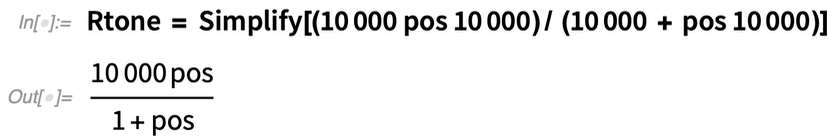✕

The relation between the equivalent resistance and the position of the knob looks as follows. Using that value, we can easily calculate the cutoff frequency of the RC filter, which is given by the formula: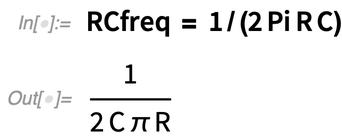✕

If we substitute the values of the components, we get the following value of the cutoff frequency in function of the potentiometer position: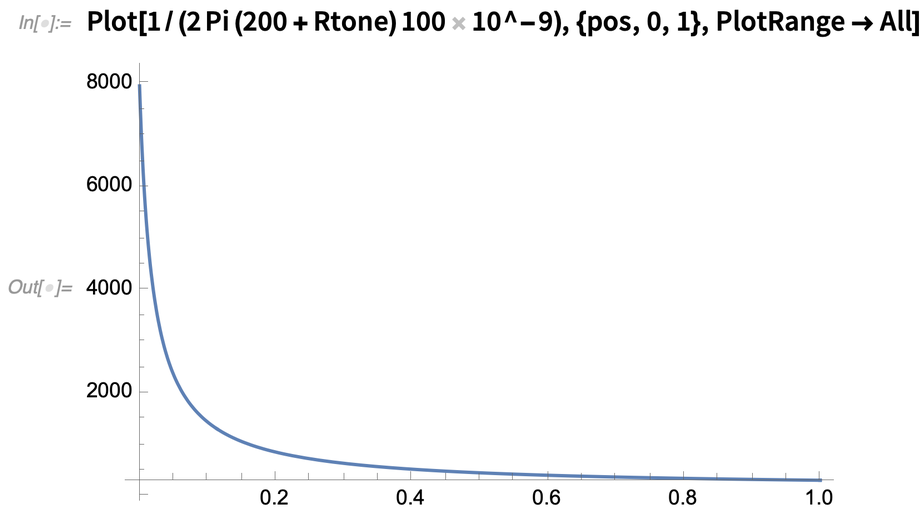✕

Now that we have a better understanding of the different stages of the circuit, let’s put them all together to simulate the full circuit: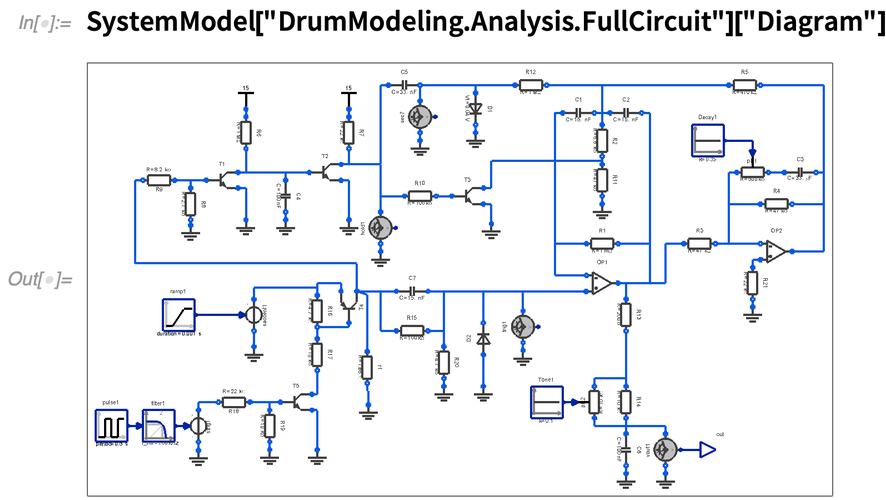✕

In this simulation, we have set the following parameters: Tone 10%, Decay 35% and Accent 8 V. As mentioned during the analysis of Section 5, we have reduced the 1 M resistor to 900 k to get a pulse closer to 4 ms. We can see the result in the following plot: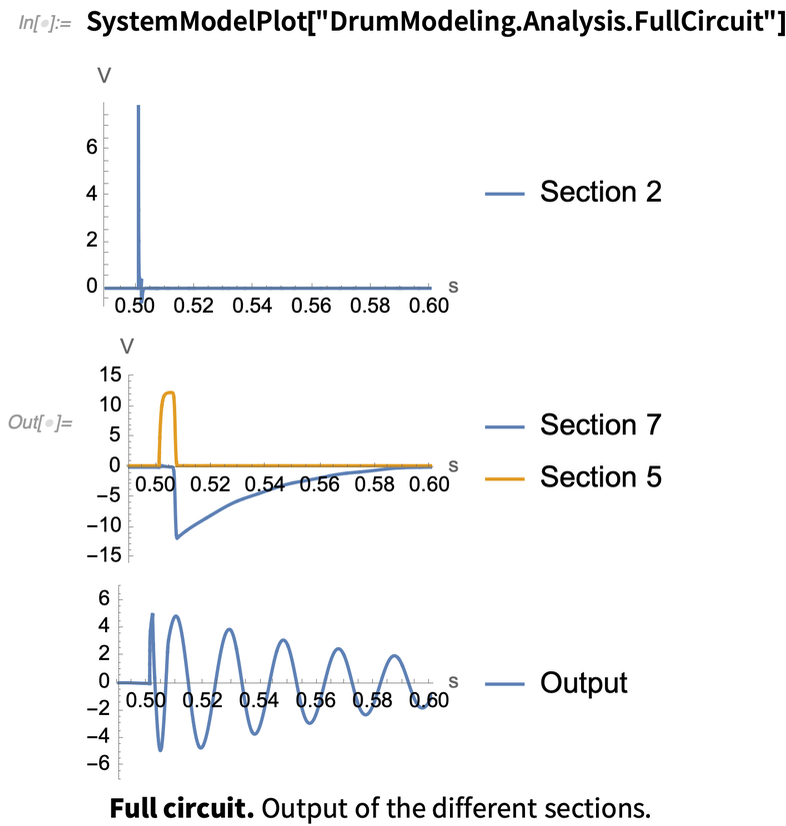✕

We can see that after the trigger, the resonator is excited by a short pulse (Section 2) whose amplitude is given by the Accent amplitude. This makes the resonator start oscillating. The 4 ms pulse of Section 5 bypasses the 47 k resistor of the resonator by short-circuiting the transistor of Section 6. The capacitor of Section 7 starts charging to +15 V. When the resonator is about to complete half a cycle (which is around 4 ms), the pulse of Section 5 goes low. This introduces in the resonator a pulse of –15 V (Section 7 output), and at the same time, the frequency of the resonator changes when reintroducing the 47 k resistor.

In summary, we can see from the output that there is a small positive “click” followed by a negative half-cycle of a sine wave. This half-cycle is about twice the base frequency of the resonator. After that half-cycle, the resonator oscillates at the base frequency until the energy dissipates according to the set Decay.

We can listen to the sound produced by this simulation in the following audio output. (Again, because it is a low frequency, you may need to play it using a decent sound system.)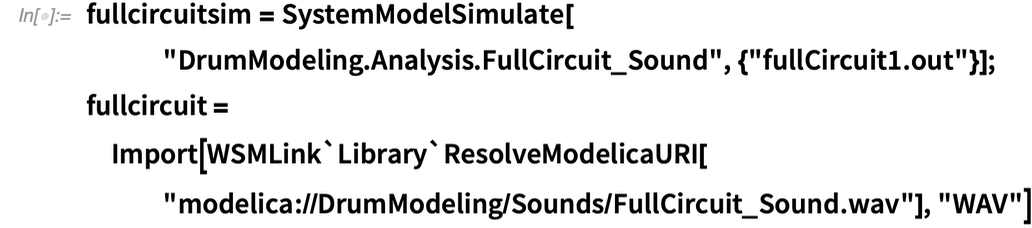✕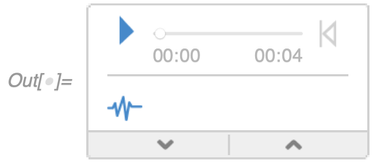• It contains 160 equations.
• It requires solving 7 linear systems of equations and 1 nonlinear system.
• It simulates 7 semiconductor components (5 transistors and 2 diodes).
• On my computer, it takes 3.5 s to run a simulation of 4 s.

In our previous analysis, we discovered that practically all semiconductors in the circuit are used as switches. We could create a more efficient model by simplifying all the circuit sections according to their behavior. But before doing that, let us take a look at how this model matches our real kick drum.

## Comparing to the Real Circuit

In the beginning of this post, we saw a picture of the circuit I built to use as a reference. There are a few things to consider when comparing the model against the real circuit.

First, my circuit uses resistors with a tolerance of 1%, while some of the capacitors have 5% and others 1%. My replica of the drum is powered by ±12 V coming from my Eurorack power supply, while the original drum machine used ±15 V. This difference may have a small impact on Sections 5 and 7, according to our analysis. But these differences can be compensated either in the simulation model or in the real circuit. Finally, I used general-purpose transistors that do not match the ones used in the original drum machine, but because they are used as switches, I expect the differences to be minimal. It is important to note that in the real circuit, it is hard to set the exact values of Tone and Decay, and this may have a small impact on the measurements.

In the following image, you can see the measurements taken from the input Trigger (blue) and the pulse of Section 5 (yellow). You can see that the total length of the pulse is around 6.2 ms. This number is very close to the 6.3 ms of our simulation. These numbers are close, however, to each other for different reasons. As mentioned before, this circuit is powered with ±12 V, which will make the capacitor of Section 5 charge slower, therefore making the pulse longer. The transistor model needs a slightly larger voltage to turn on; therefore, the pulse is longer: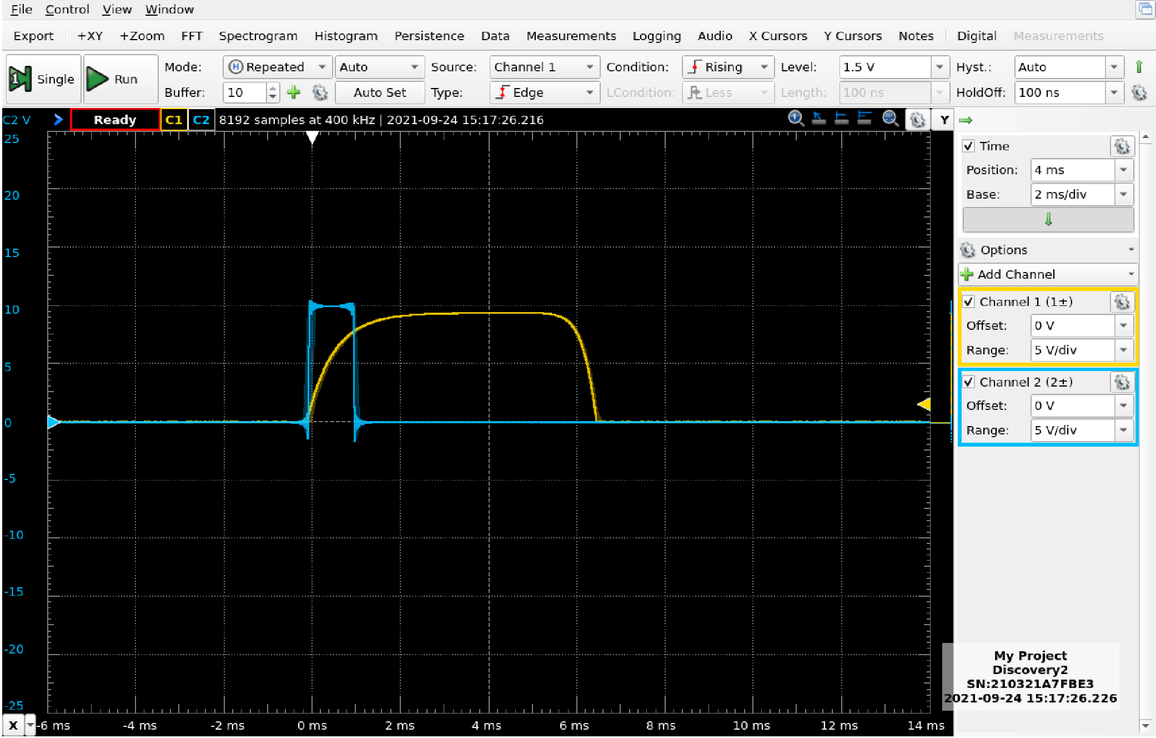✕

In the next screenshot, we can see the output of the drum (blue). We can see in this measurement that during the 6.2 ms, the resonator almost completes half a cycle. The 6.2 ms end when the resonator is close to the positive peak. This implies that the resonator is oscillating faster than the simulated resonator: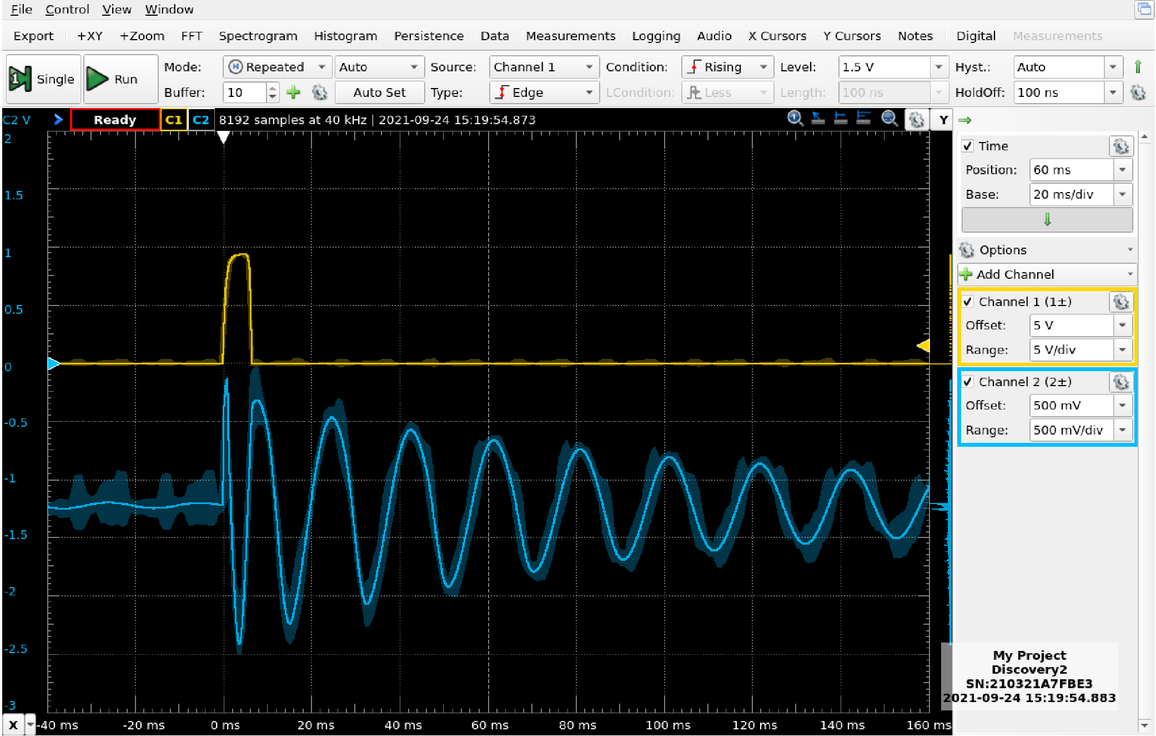✕

In the following plot, we can see the measured drum sound (blue) and the simulated sound (orange) side by side: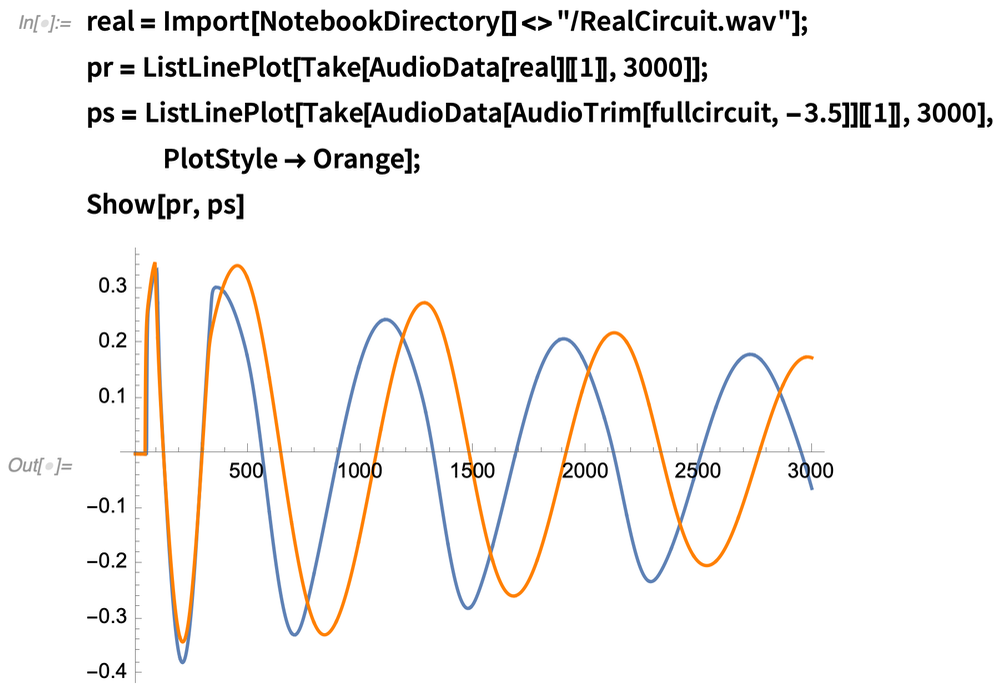✕

In this plot, it is easier to see that the real resonator oscillates faster than the simulated one. Now let’s listen to the sounds: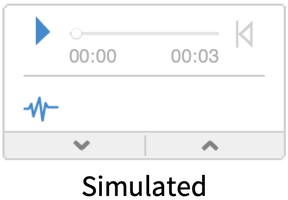If you have a good ear, you may notice that, indeed, the real drum sounds slightly higher in pitch. Apart from that, they sound very similar.

At this point, there are a few paths that we can follow:

• Tweaking the model so it matches the real drum.
• Fixing the real drum so it matches the service manual (making the pulse 4 ms).
• Making the model match the service manual by adjusting the frequency and fixing the 4 ms pulse.

For this example, I will try to make the model match the real drum by tweaking some of the parameters. After changing the Tone and Decay parameters and modifying the resistor values of the resonator, I got the following sound: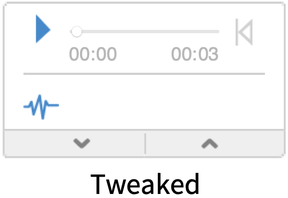This tweaked model is a better match. There are still a few improvements that we could make to our model if we would like to get a better matching of the waveforms. For example, in the real circuit, the resonator frequency seems to change more gradually, so it is faster in the beginning until it settles in the main frequency. Our simulation model settles sooner. This could be caused by the nonlinearities of the transistors or the op-amp. This behavior could be simulated by tweaking the transition curve when switching the resistor in the resonator on or off. Based on the previous results, however, the sound of our model is close enough.

In the next section, we are going to try to simplify our model even further and also try to improve it based on the observed behavior.

## Simplifying the Simulation Model

As already mentioned, the simulation model presented can be optimized by using simplified models of the components. We can also take advantage of the power of the Modelica language in System Modeler to create simplified behavioral models that match the behavior of the original circuit. The objective of this is to create a simpler and faster model that we can port to an audio platform and use to create actual music. In my previous blog post in this series, I showed how I create plugins for the VCV Rack open-source Eurorack simulator.

Because we already have the circuit split into sections, we can try to create an equivalent simplified model for each section. We’ll use causal connectors to communicate each of the sections of the circuit. This means instead of using electric terminals (acausal connectors), we will use inputs and outputs.

Let’s start with Section 1. When analyzing this section, we concluded that it behaves as a controlled switch. The Trigger signal, which can be represented as a Boolean signal, controls the flow of the Accent signal. Using System Modeler, we could replicate this behavior by using some of the existing components. In this case, however, I have decided to create my own model to show you how to make custom models using the Modelica language.

I start by creating a new model in System Modeler. (Check out the System Modeler tutorials to learn more about model creation.) Then I drag the inputs and outputs into the Icon View to locate them in my preferred position: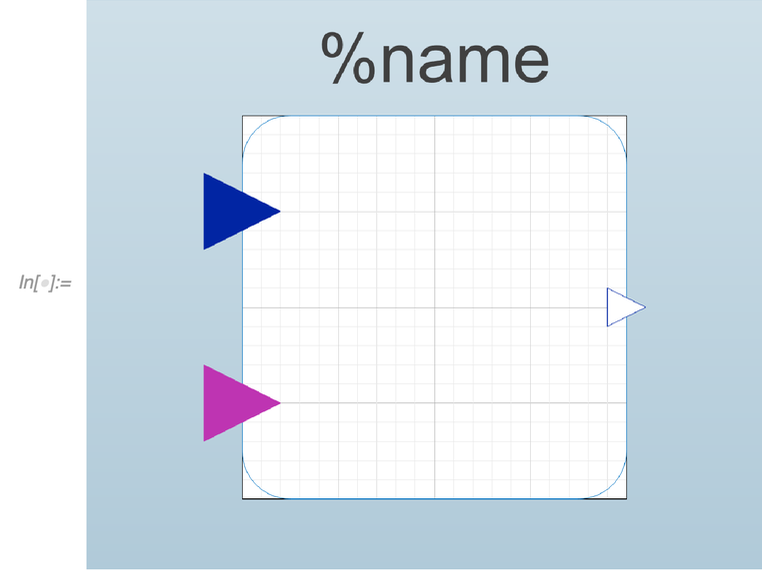✕

Once I have the icon for my model, I can switch to Text View to type the code of my behavioral model: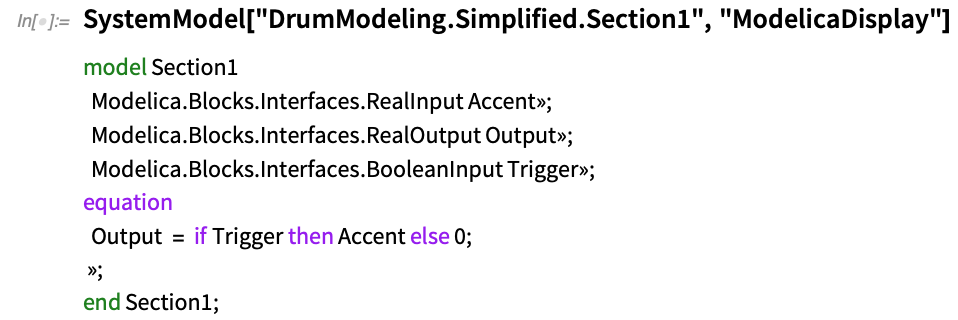✕

You can see in the code that this model consists of a single equation that defines the value of the output. You may notice that as soon as the Trigger signal becomes “true,” the Accent is sent to the output. This model differs from the original when the Accent signal is less than 2 V. In that case, the original model did not produce an output. This is not an issue because the Accent signal is supposed to have a range from 4 V to 14 V. On the contrary, this simple model would allow us to produce sounds with an Accent less than 2 V. In the following plot, you can see the results. Modeling it this way removes two transistors: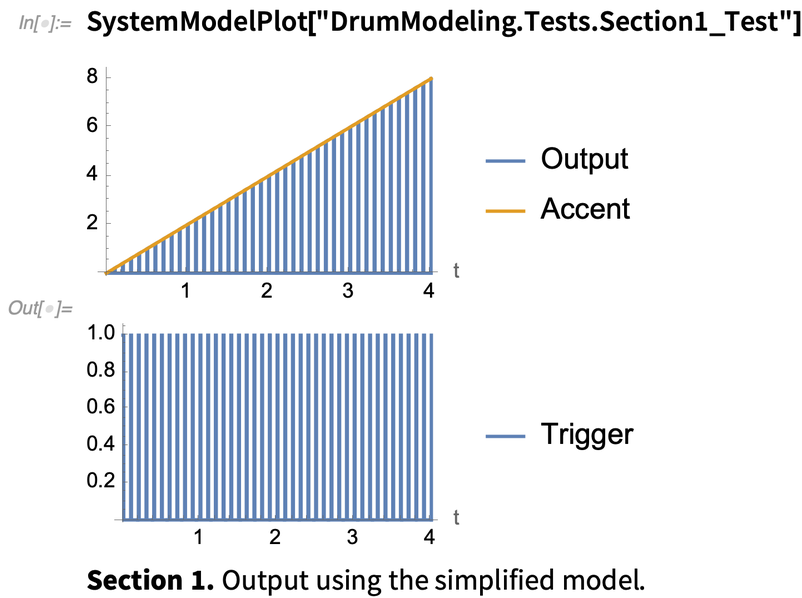✕

Continuing with Section 2, this model is easier to create by using existing components. If you recall, this section used a diode to suppress the negative peak produced by the highpass filter. We can create an approximated behavior by removing the diode and adding a limiter at the output: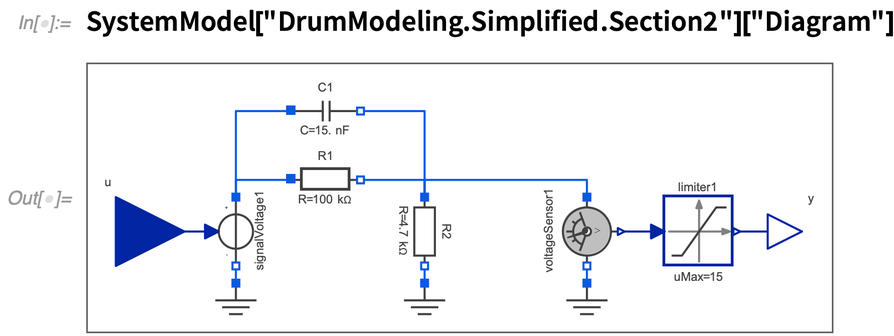✕

Compared to the original model, this simplified version completely blocks the negative output. The biggest difference is that the capacitor is not being discharged by the diode. Therefore, if a new trigger comes before the capacitor is discharged, the output will be slightly different. This is not a big issue since we do not expect to have two triggers in less than 300 us, which is the time the capacitor takes to discharge: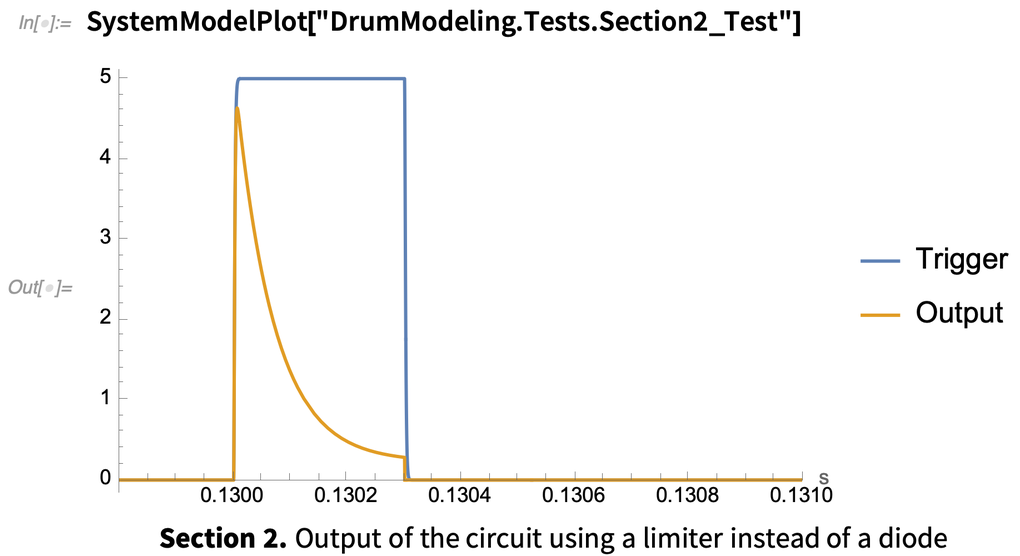✕

By removing this diode, we have removed from the complete model the only system that required a nonlinear solver when simulating.

Before going into Section 3, we are going to continue to Section 5.

Section 5 is in charge of producing a 4–6 ms pulse for every Trigger. We can easily describe this behavior with a snippet of Modelica code: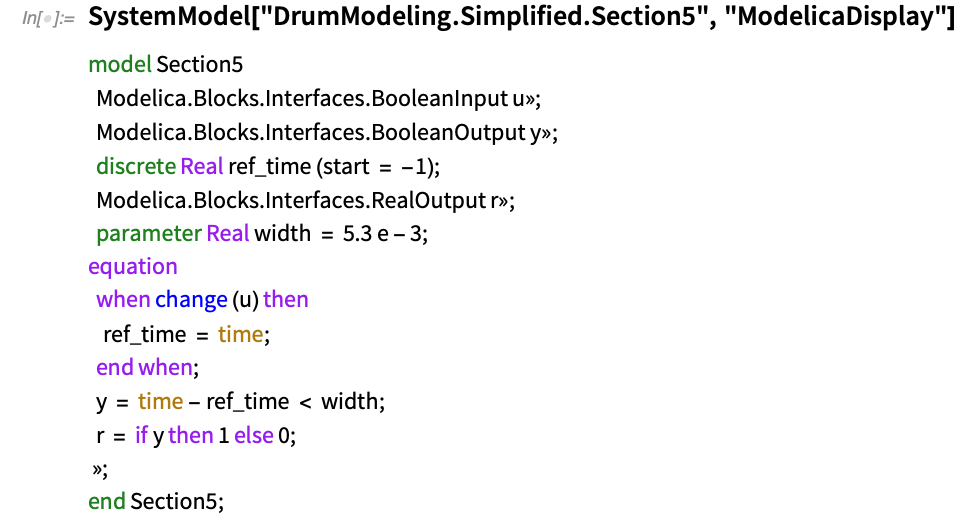✕

This simple model produces the pulse and outputs it in two ways: one as a Boolean signal and the other as a Real. In this specific case, the pulse is 5.3 ms to match our previous simulation model. By using this simplified model, we get rid of two more transistors.

Section 7 is modeled using a combined graphical and textual model. In this graphical model, you can see one capacitor and a single resistor. The resistor acts as a load and discharges the voltage of the capacitor: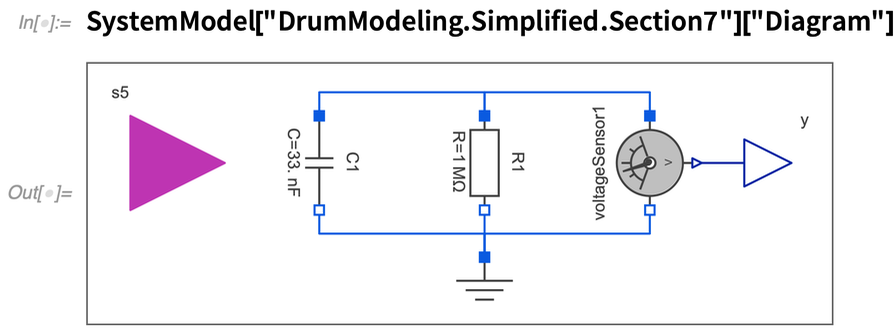✕

The capacitor is charged to –11 V when the signal of Section 5 (the 4 ms pulse) ends. To describe that behavior, we use a “when” equation in Modelica. This equation reinitializes the state of the capacitor to the target voltage. Here is the code part of this model: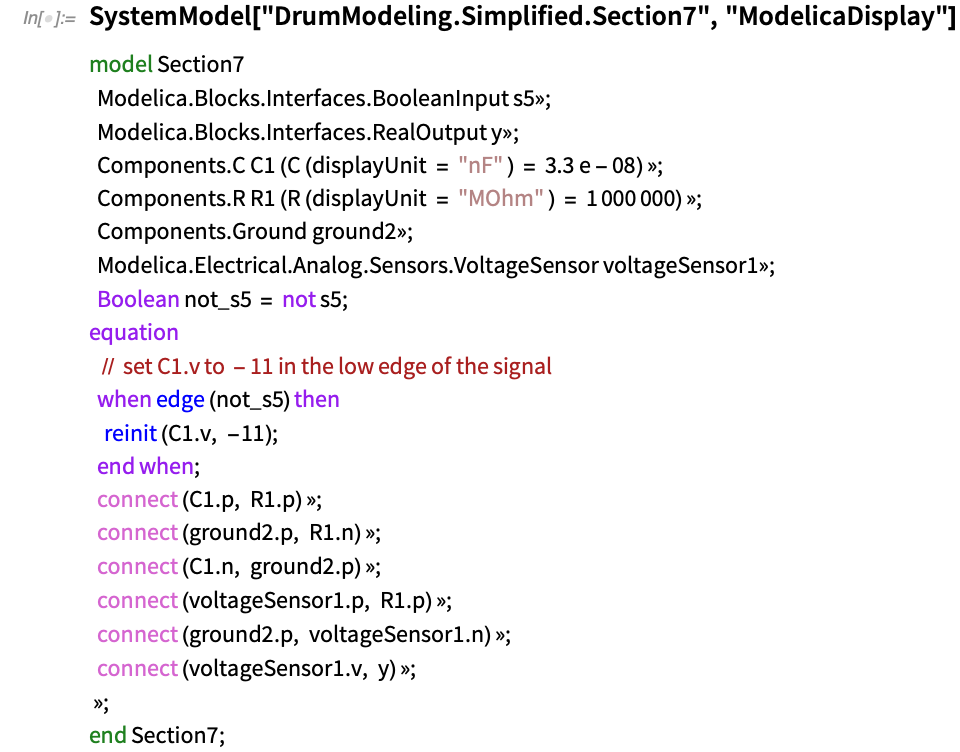✕

By making this change, we get rid of the second diode. In the next plot, you can see the results of the behavioral models we created for Sections 5 and 7: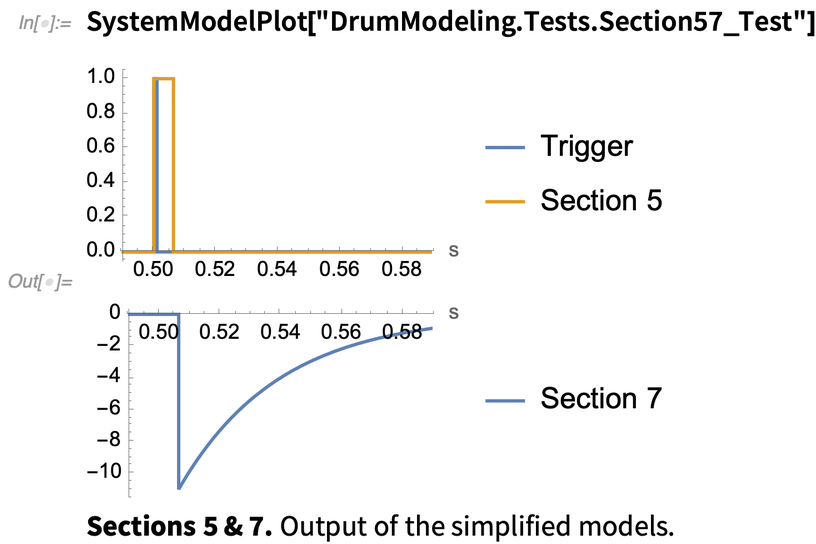✕

Now let’s go back to Section 3. If you take a look at the following diagram, we have combined Sections 3, 4 and 6: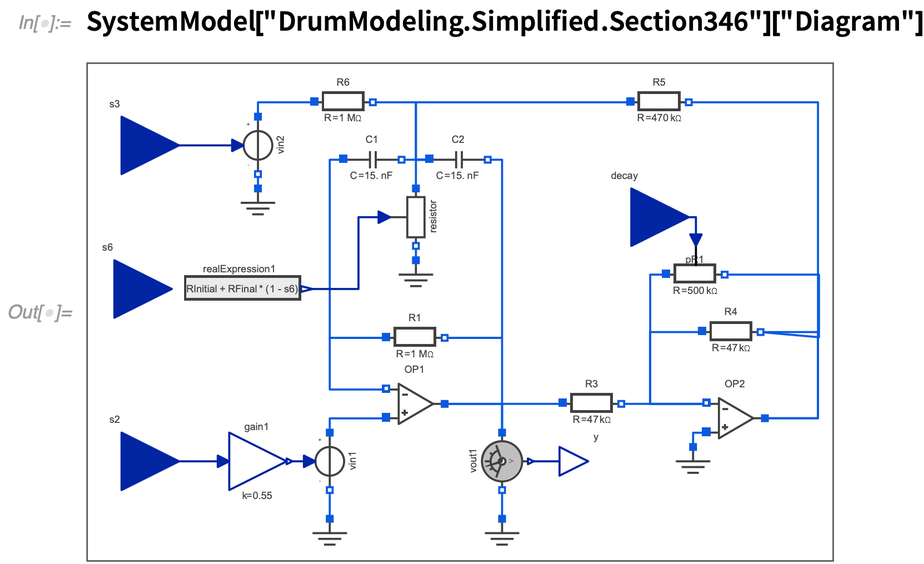✕

The transistor used in Section 6 to change the oscillation frequency has been replaced by a variable resistor that takes the Section 6 signal and uses it to define the corresponding resistor value. The capacitor in Section 6 that was used to let the DC pass unaffected is removed.

Finally, Section 8 is very similar to what we had before: a simple lowpass RC filter. You can see it in this diagram: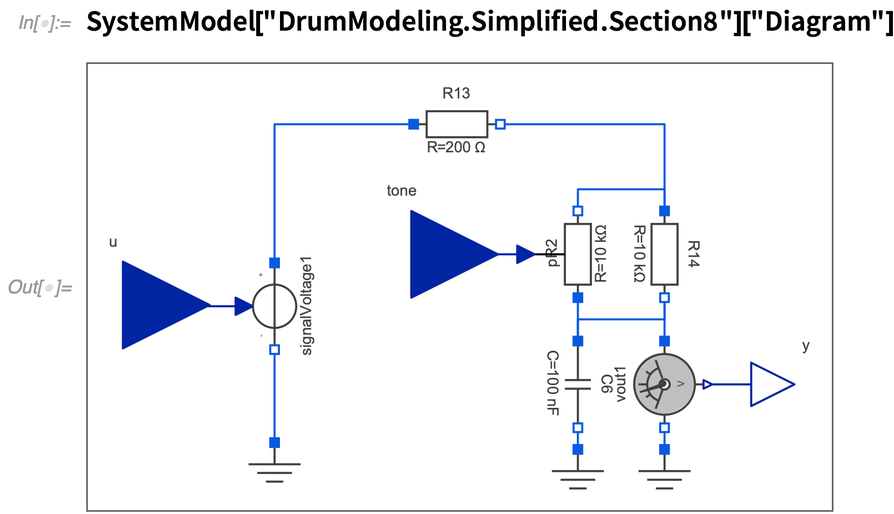✕

When we put all the simplified models together, we end up with the following simplified model: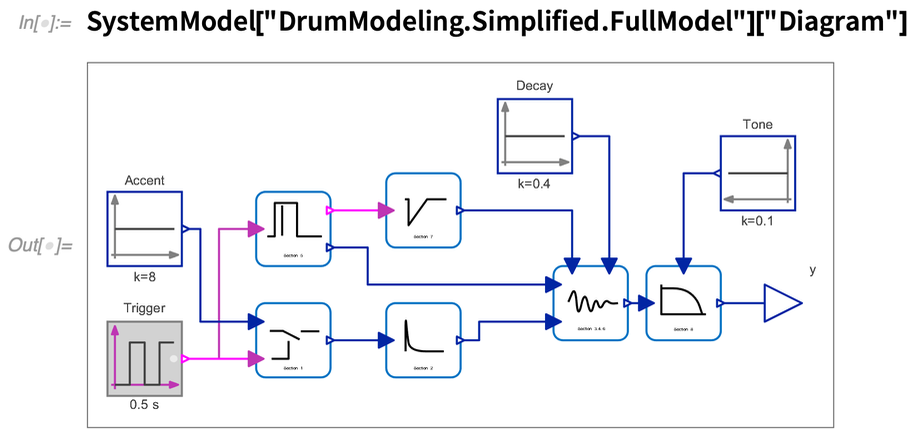✕

Before seeing the result of the new model, let’s take a look at some insights:

• It contains 55 equations (compared to 160 in the original model).
• It requires solving 3 linear systems and 0 nonlinear systems.
• When simulating 4 s, it takes 0.35 s (almost 10x faster than the original).

This plot shows the results: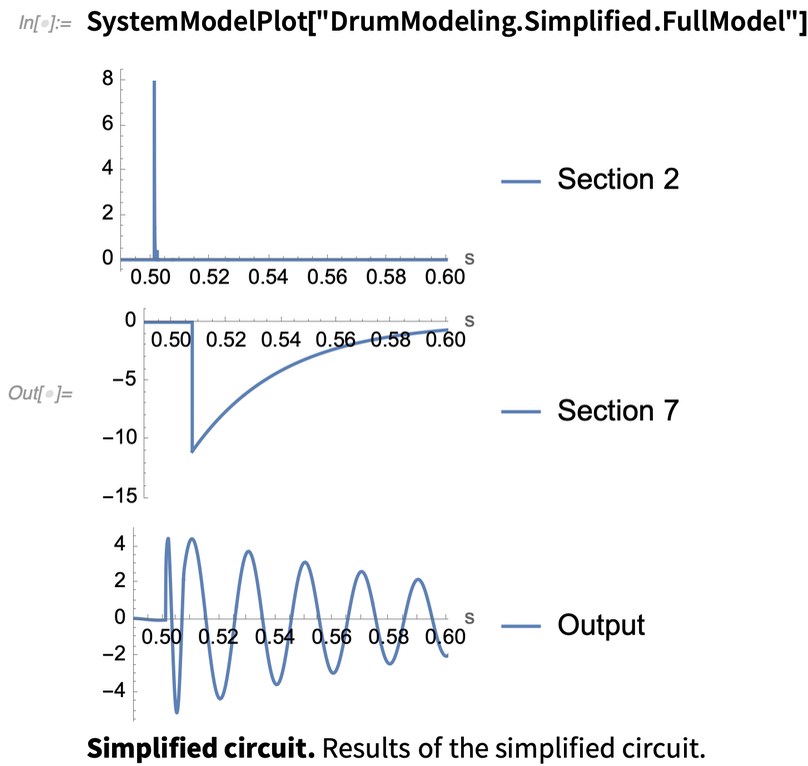✕

When looking at the output signal, it is hard to spot the differences. Listen to both sounds:Both models sound extremely similar. In a blind test, it would be hard to determine which one is more accurate and which one is simplified. If we look at the simulation results, however, we can spot small differences. Here, the original model is in blue and the simplified in orange: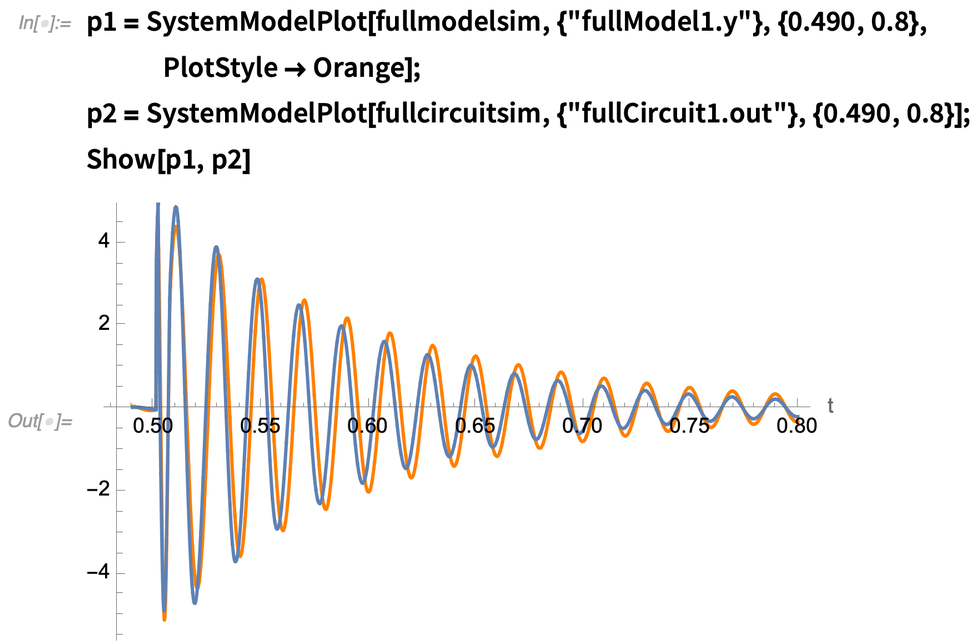✕

Here is a close-up of the initial transient: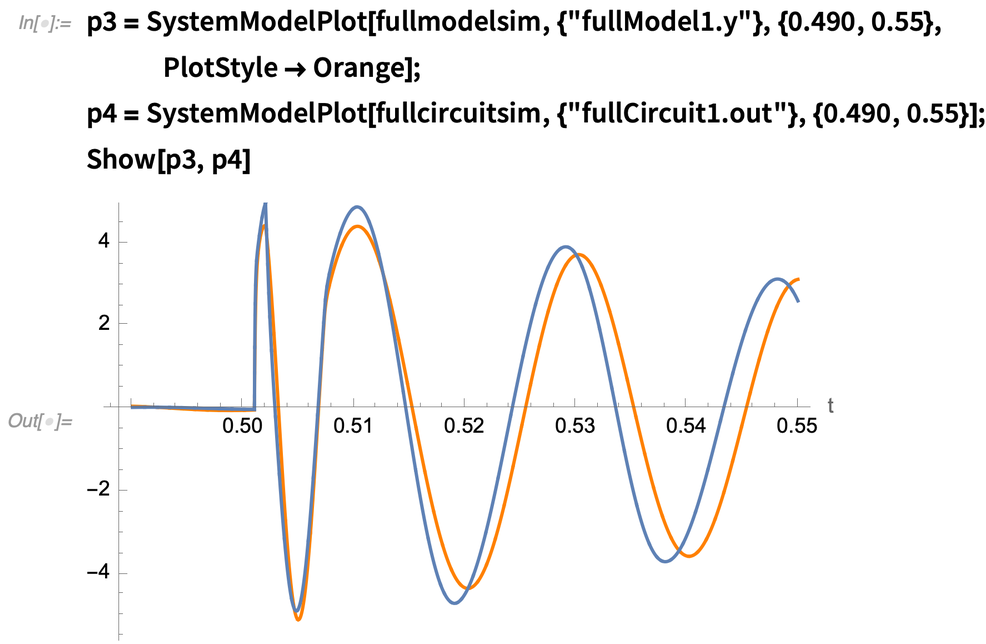✕

I tweaked a few parameters in the simplified model to better match them, including the value of the main resistor of the resonator, and added a few gain blocks. Some of the differences in the results may come from the fact that we replaced all the transistors with ideal switching devices. We removed one capacitor in Section 4. In addition, all our sections are completely decoupled from each other, while in the original circuit, because of the nature of electric components, one section can affect the behavior of others though the current flow.

When we compared the original model against the real drum, we mentioned that the real resonator did not make an immediate transition to the base frequency. In the simplified model, we can replicate that behavior by changing the Section 3 resistor value gradually, for example by using a lowpass filter. In addition, I inserted a gentle nonlinearity at the output to limit the amplitude of the initial peak.

Listen to the real drum and improved model: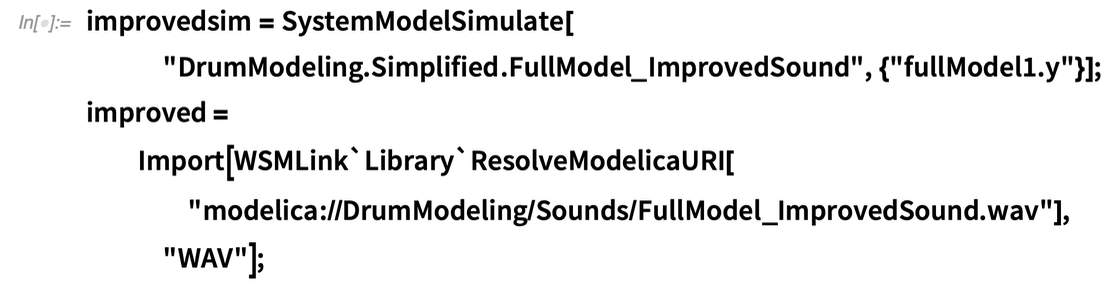✕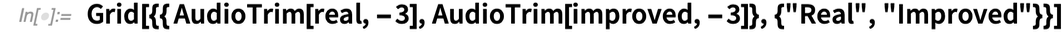✕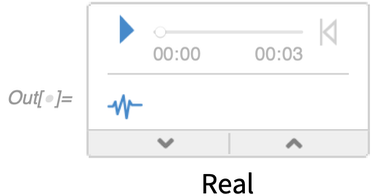The following plot shows a comparison of the waveform produced by the model and the real drum: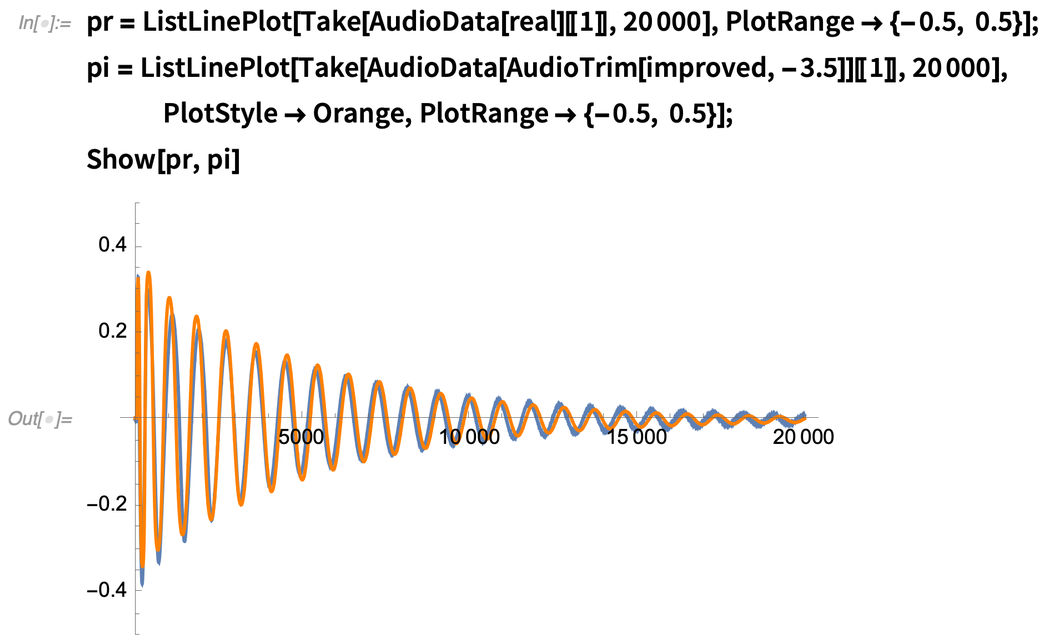✕

You can see in the previous comparison that, overall, this model is a good match to the real drum. It still fails to reproduce, however, all the effects in the initial transient: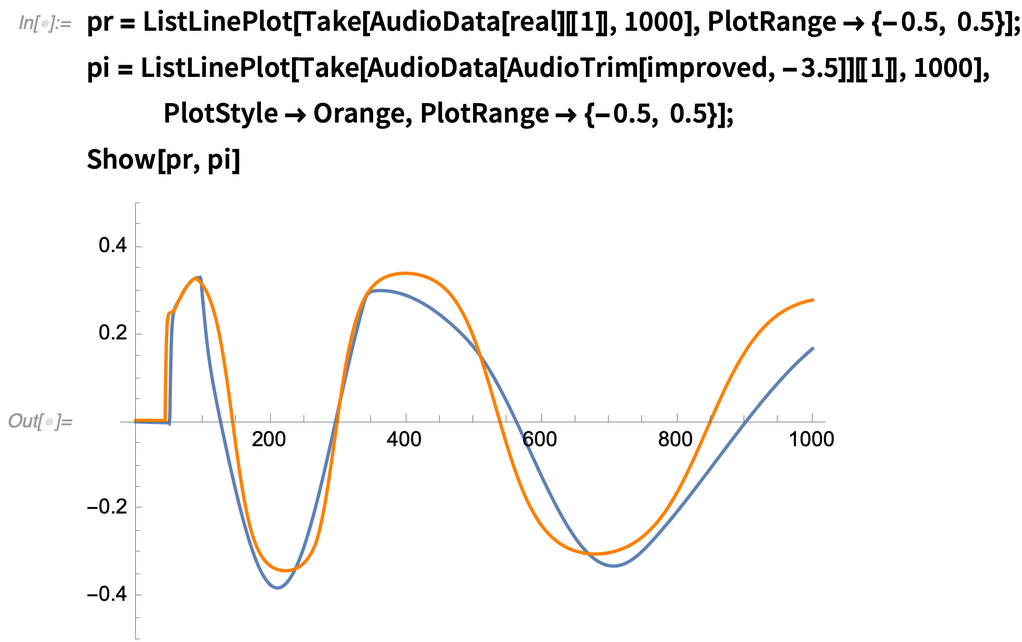✕

At the end, the selection of the model variations presented in this post depends completely on the application. If we want to run these models to consume as little CPU as possible, we can further simplify them. If we want to achieve full realism of the sound, it is at the expense of computational power.

In summary, the simplified model is a good approximation to the full circuit, and it can be tweaked to improve the matching with either the real hardware or the complete simulation model.

## Extending the Basic Functionality

Based on our analysis, we were able to find a few components whose values may have a significant effect on the sound. We could replace these fixed components with variable versions to expand the sonic capabilities of the drum. For example, we could change the resistor setting the pitch of the drum by a potentiometer (see the next figure). That would allow us to change the pitch of the drum. A value of 50 k will give us approximately one octave of range: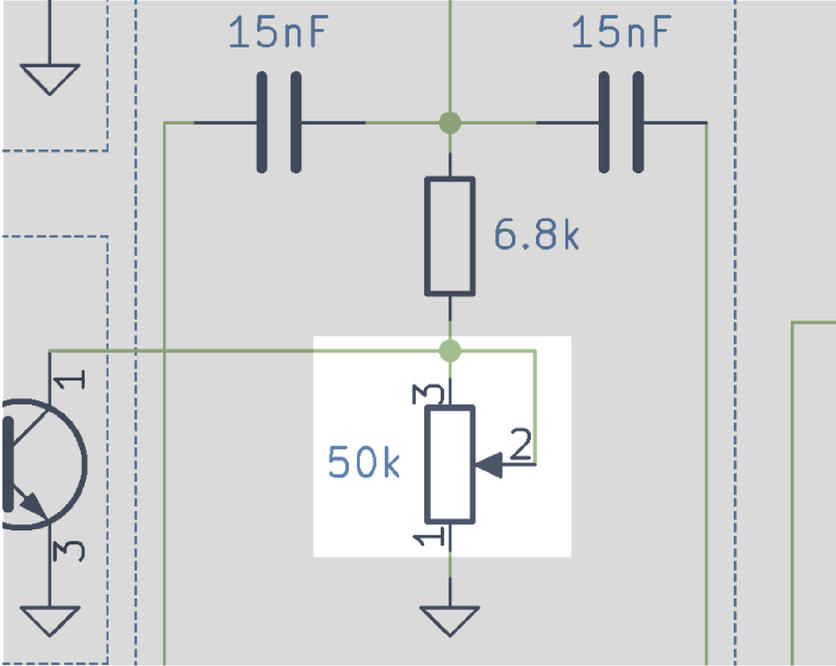✕

Other possible modifications would be to change the length of the Section 5 pulse, but we are not going to cover it here.

The following is a demo of the sounds that could be produced if we changed all the parameters of the drum (including the pitch):✕✕## Optimizing the Model for Real-Time Execution

Implementing the simplified model is still a nontrivial task. There are some sections, like the main resonator, that we have described using electric components. As in my previous blog post, the model would be easier to implement in a language like C++ or Vult if it is in ODE form. We are going to see how this can be done for the main resonator. The other sections are easier, and a similar approach can be followed.

The component for the main resonator is made in such a way that it uses only linear components. This will make it easier to reduce the equations to a minimal form by using the functions provided in the Wolfram Language to manipulate System Modeler models: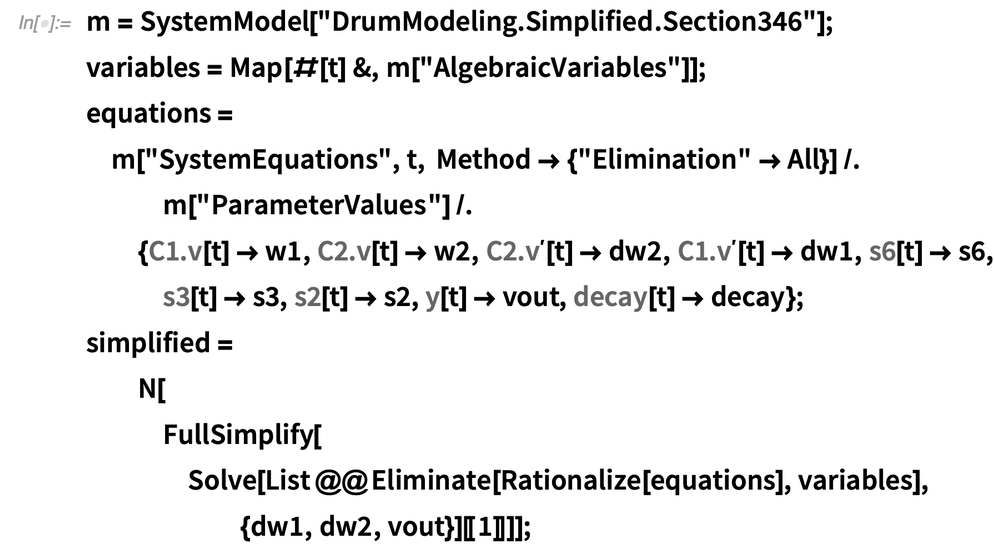✕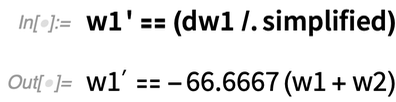✕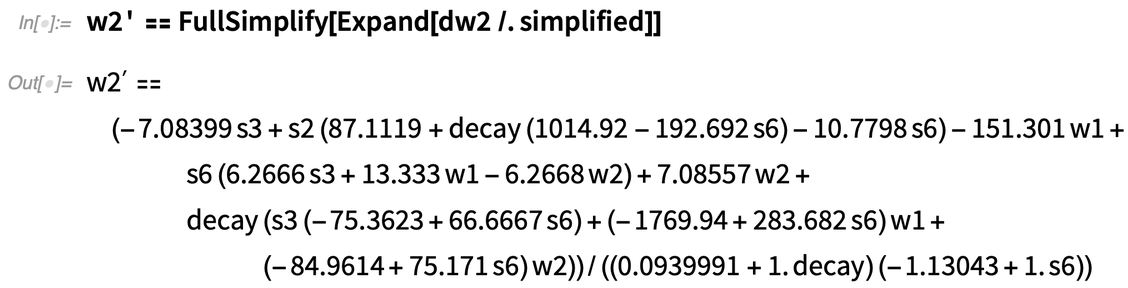✕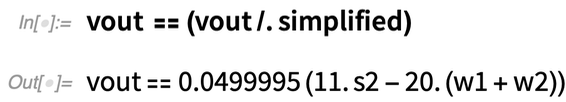✕

These three equations are easily translated to the implementation language. In this blog post, we are not going to cover the implementation process, but you can refer to my previous post, “Digital Vintage Sound: Modeling Analog Synthesizers with the Wolfram Language and System Modeler.”